# Curriculum for Pre-kindergarten

Students lay the foundation of number sense and mathematical thinking as they progress through very concrete, hands-on exercises that prepare them for future success in math.

## MODULE 1 Counting to 5

### Topic A: Identifying Attributes

Students learn the meaning of same, different, alike, larger, and smaller. They identify and match objects based on size, color, shape, and even purpose.

#### Identify two out of three objects that are the same

Build an understanding of the word "same." The identify which two objects are the same when shown three objects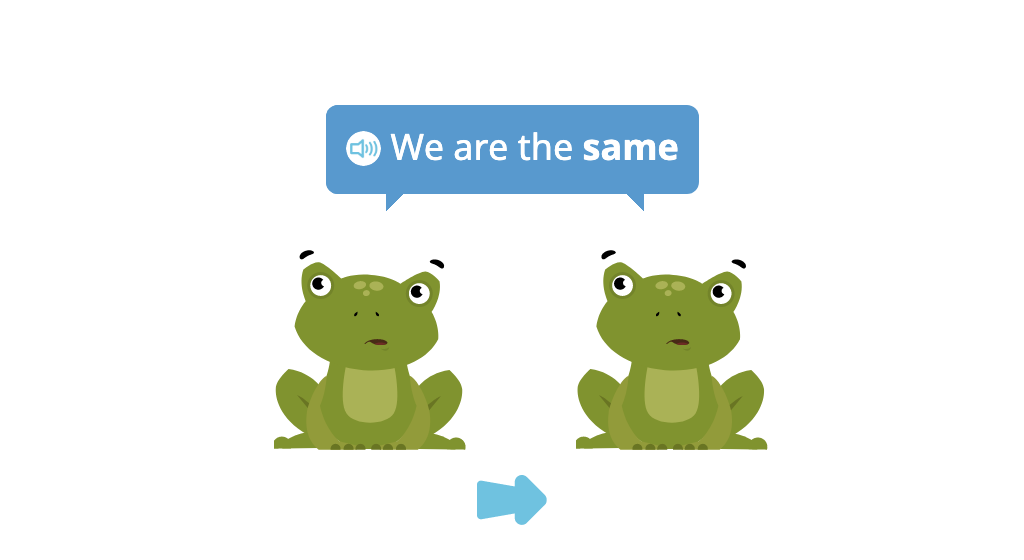#### Identify two out of three objects that are different

Practice the difference between "same" and "different." Then look at three objects and choose two objects that are different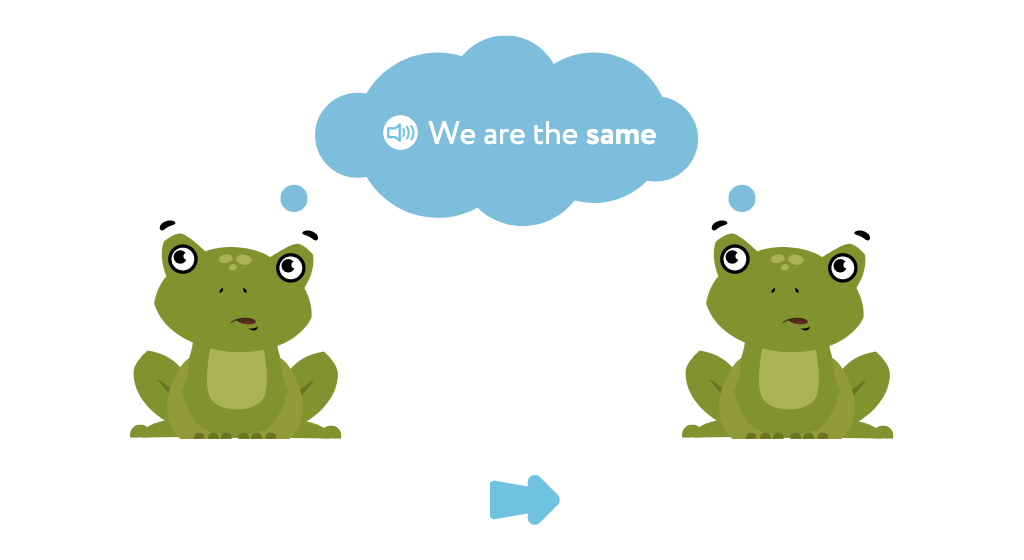#### Identify whether two objects are the same or different

Practice comparing objects. Look at two objects and tell whether they are the same or different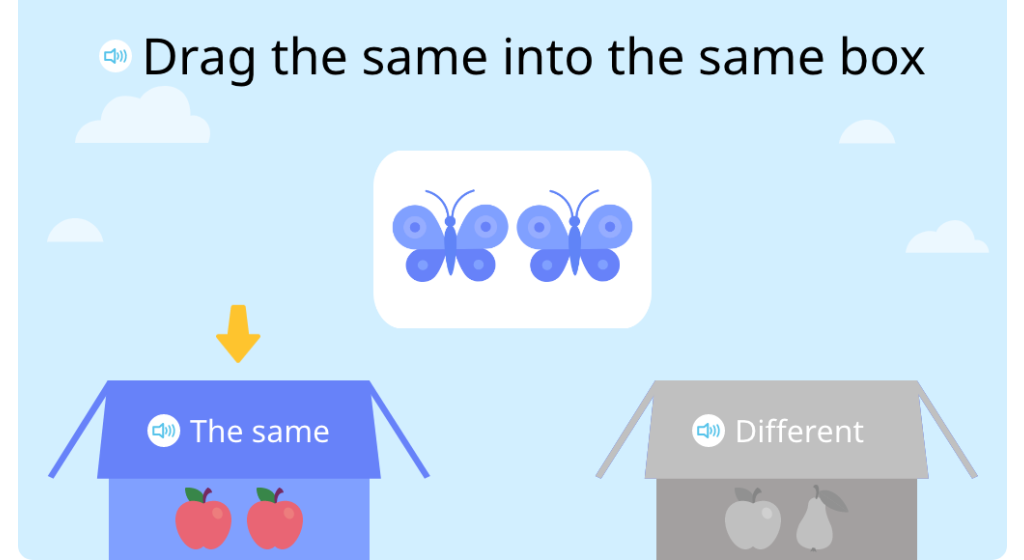#### Identify which of two similar objects is larger

Practice comparing objects. Look at two objects that look alike but are different sizes. Tell which one is larger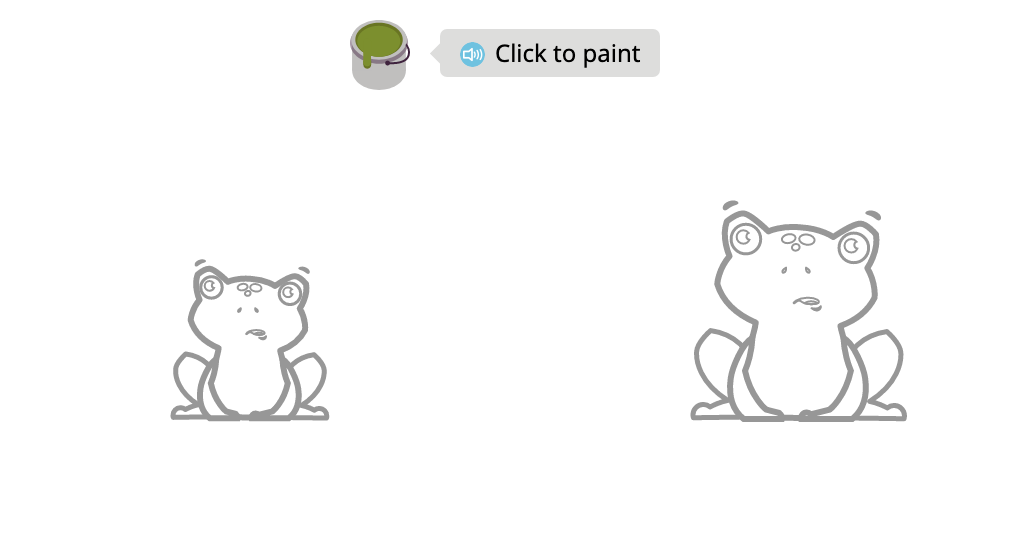#### Identify which of two similar objects is smaller

Practice comparing objects. Look at two objects that look alike but are different sizes. Tell which one is smaller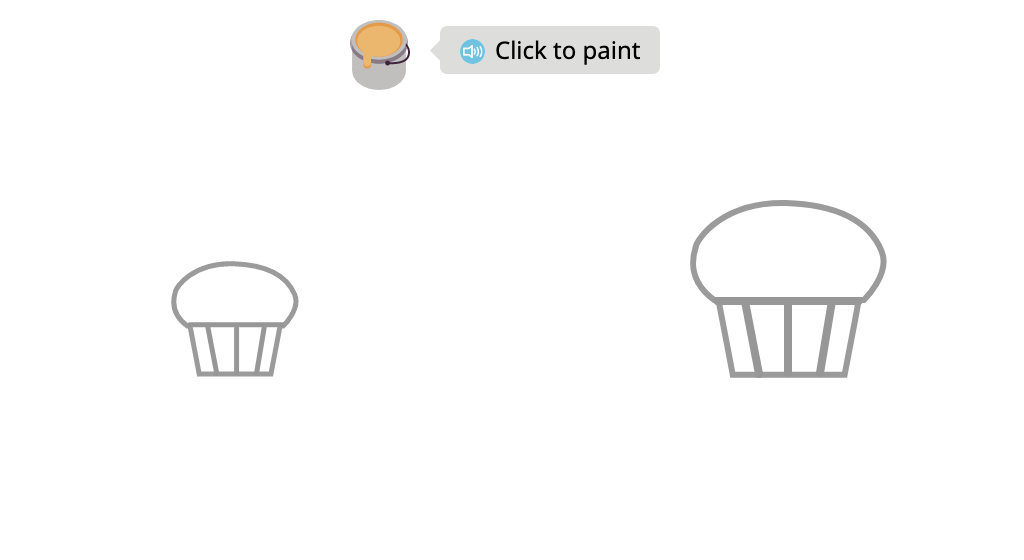#### Match pairs of similar items that differ in size and/or color

Match pictures that show the same object. The objects may be different sizes or different colors, but are alike because they show the same object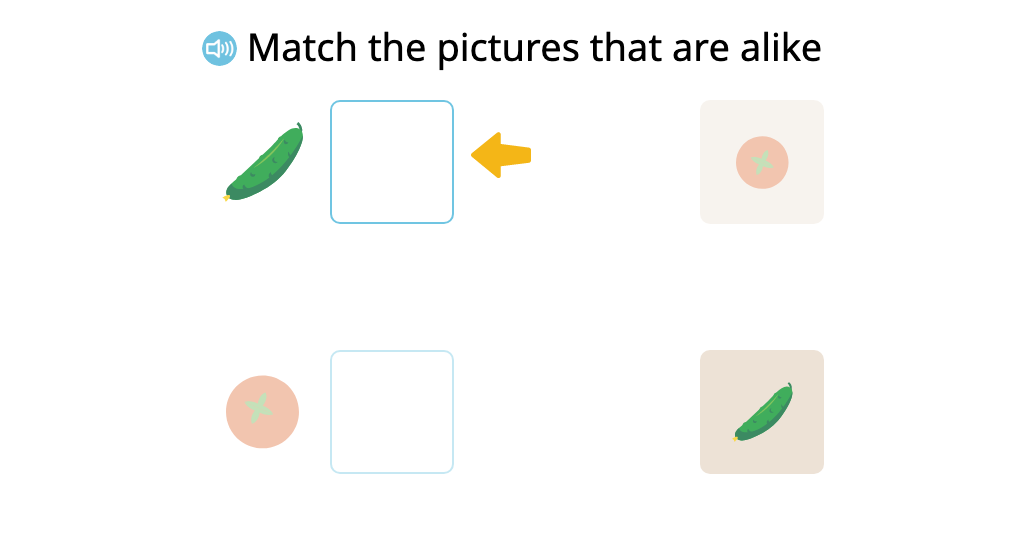#### Match pairs of similar items that differ in size and color

Match pairs of similar objects that differ in size and color. Look at pictures and match the pictures that show the same object, even if the color or size is different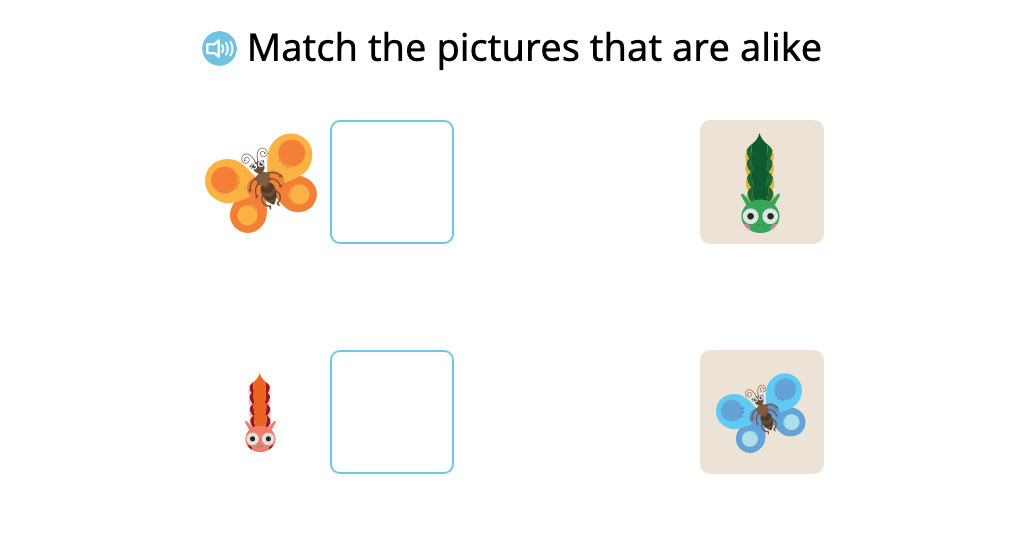#### Match pairs of different items that are used together

Match pairs of different objects to show which ones are used together. Instead of matching the same objects, this time you match objects based on what objects go together or what objects are used together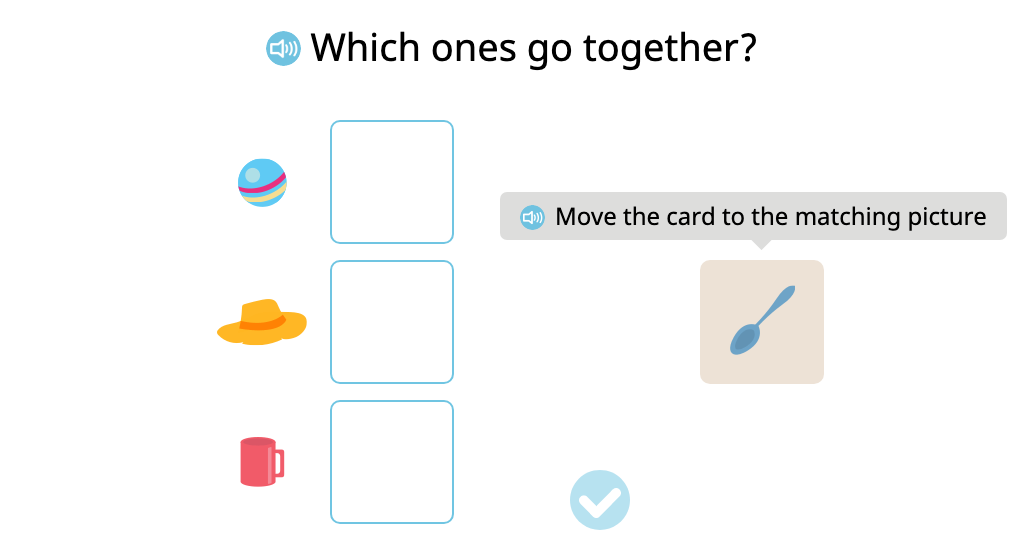### Topic B: Sorting Objects by Attribute

Students build upon their understanding of size, color, shape, and purpose to sort dissimilar objects based on a common attribute. They must also ignore objects that do not fit the given descriptors.

#### Identify objects that belong to a given category

Practice sorting objects by type of object. Feed a creature objects in a given category by clicking on all of the objects in that category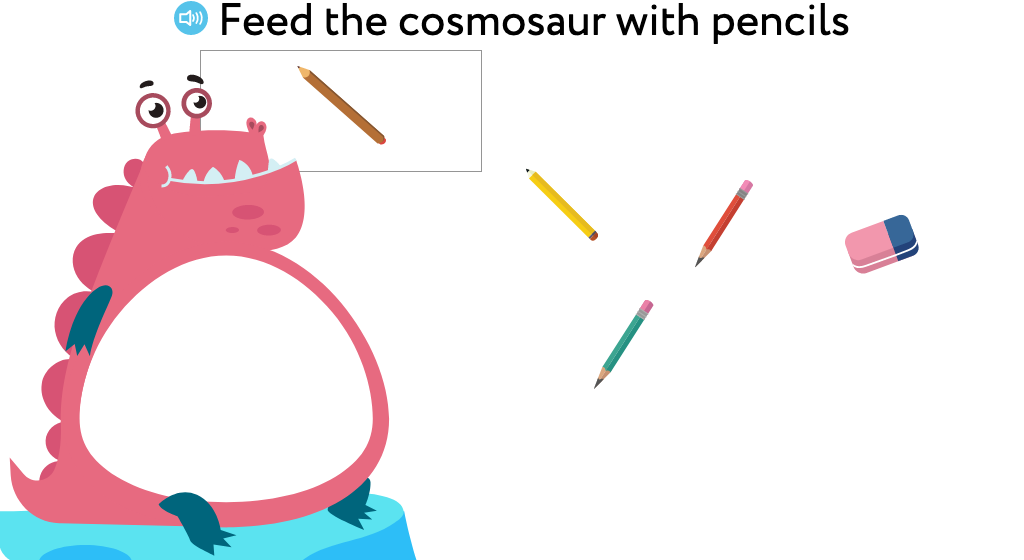#### Identify objects of a given color

Practice with sorting objects by color. Feed a creature objects of a given color by clicking them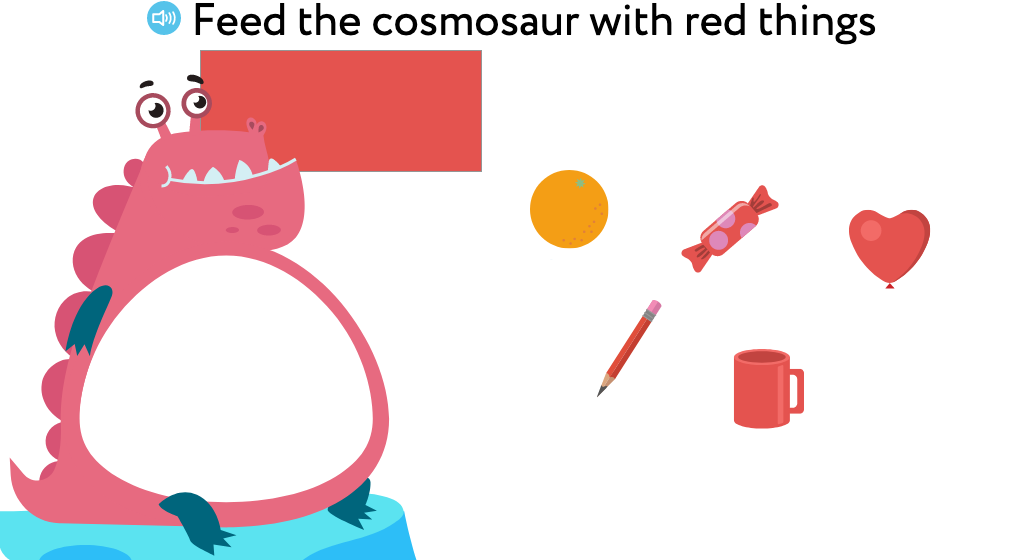#### Sort objects based on two given categories (Level 1)

Practice sorting objects by type of object. Drag each picture to the correct category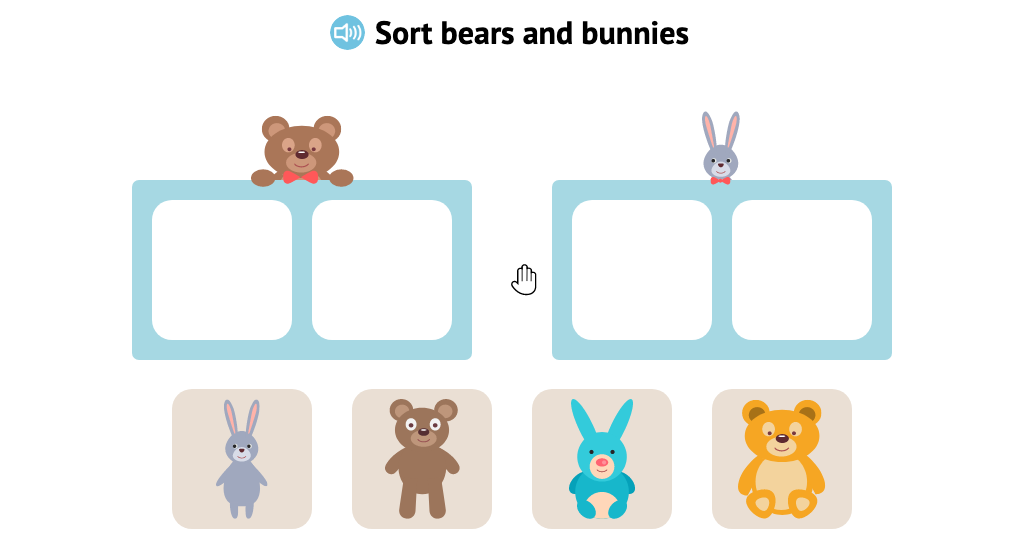#### Sort objects based on two given categories (Level 2)

Practice sorting objects. Sort the objects into two different categories. Not all objects shown are in the categories, so some objects won't be used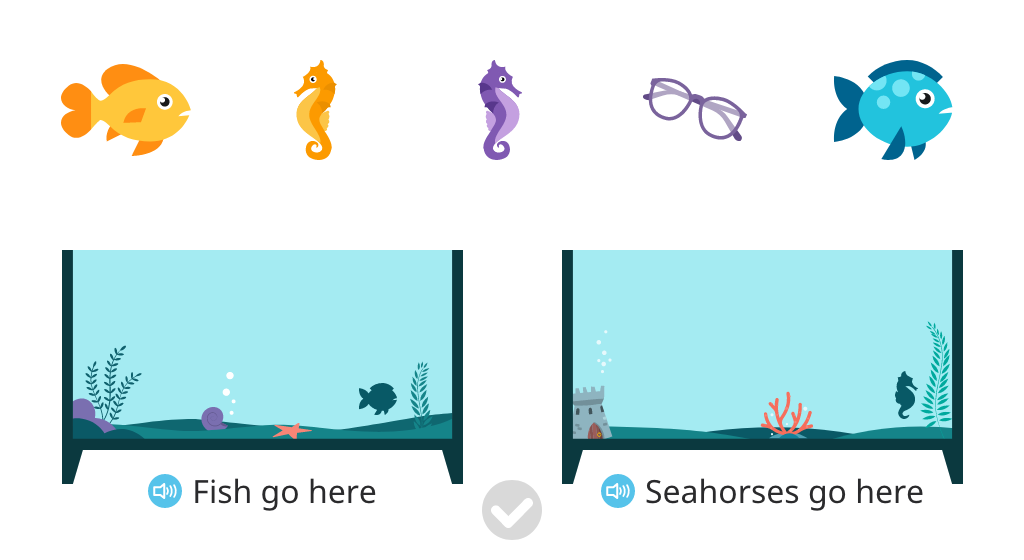#### Sort objects based on two given colors

Practice sorting objects by color. Sort the objects into two different categories based on their colors. Not all objects shown are of the given colors, so some objects won't be used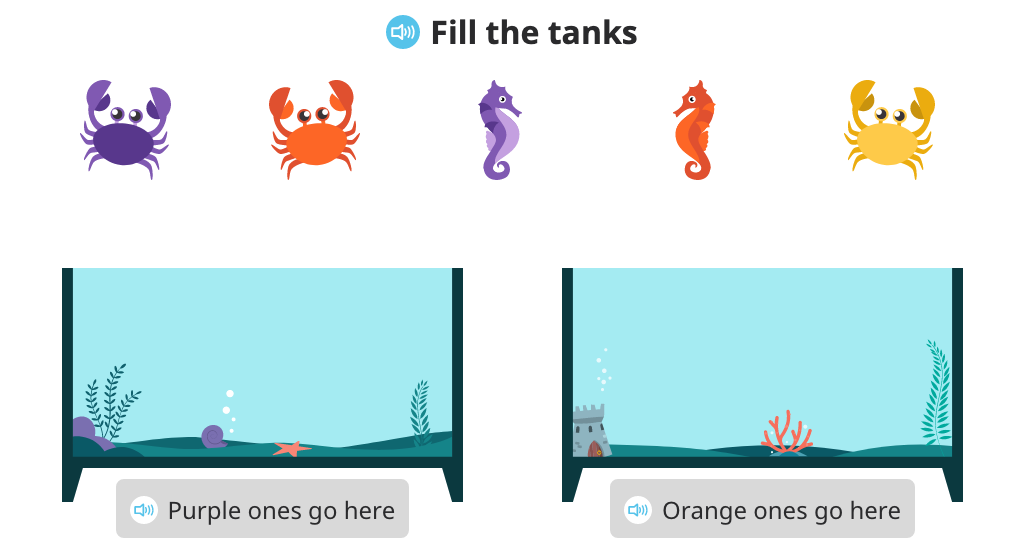### Topic C: How Many Questions with 1, 2, or 3 Objects

Students learn to count objects with 1:1 matching by interacting with each object, either by tapping it or by moving it. They work with uniform objects that are both aligned and scattered. Automatic voice prompts give directions and guidance.

### Topic D: Matching 1 Numeral with up to 3 Objects

Students match groups of uniform objects to a total written in digits. They work with both aligned and scattered objects to match objects to a number or match a number to objects. They do not necessarily count each individual object, but are encouraged to "see" the total.

#### Identify the number of aligned objects

Practice identifying the numerals 1, 2, and 3. Count up to three objects, and click on the correct number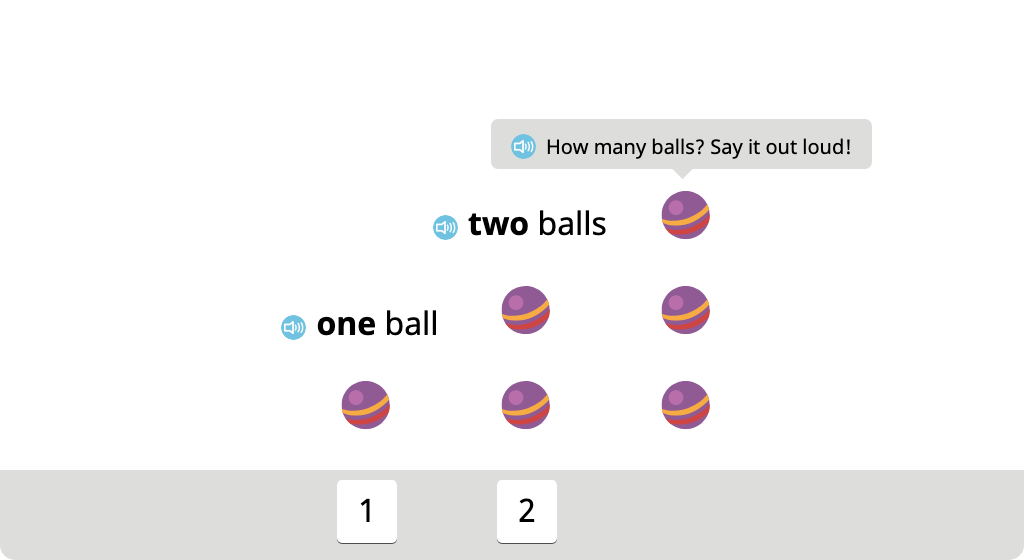#### Identify the number of aligned and scattered objects

Practice subitizing up to three objects. Recognize how many there are by sight, without counting each one. Objects may be in a row or scattered on the screen. Click the number that tells how many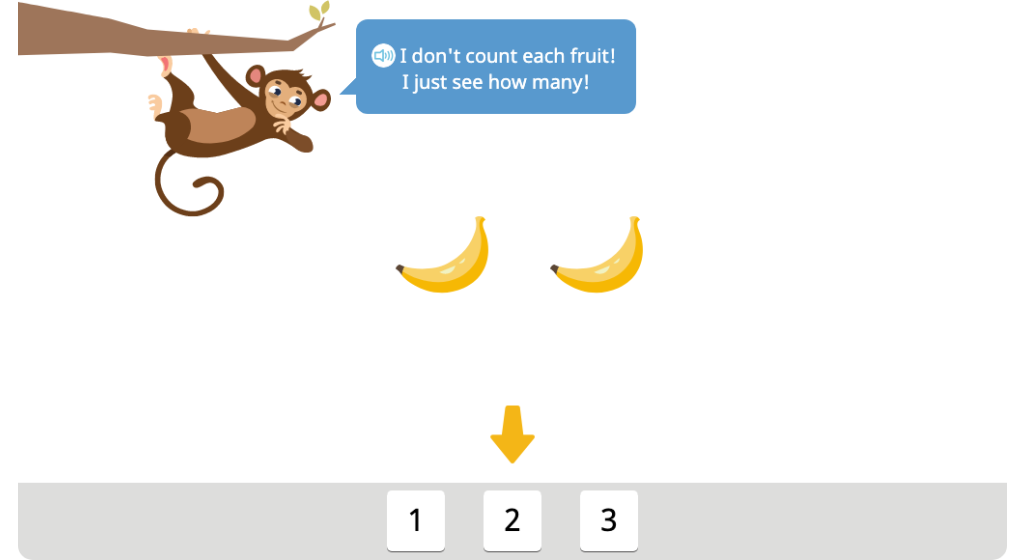#### Identify the number of scattered objects

Practice subitizing up to three objects that are scattered on the screen. Recognize how many there are by sight, without counting each one. Click the number that tells how many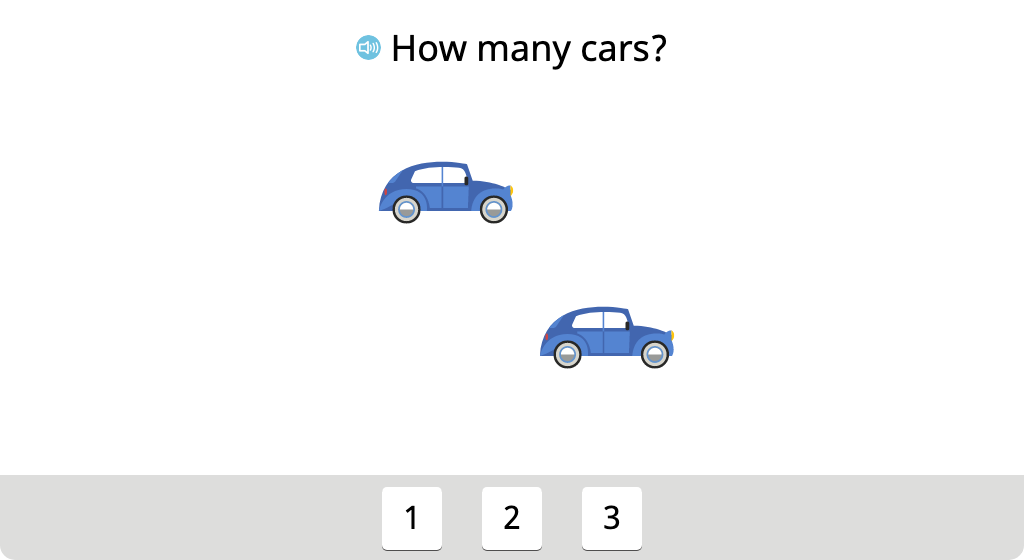#### Identify a group of objects with a given total

Look at three groups of objects. Listen to the question asking which group has 1, 2, or 3 objects. Click on the correct group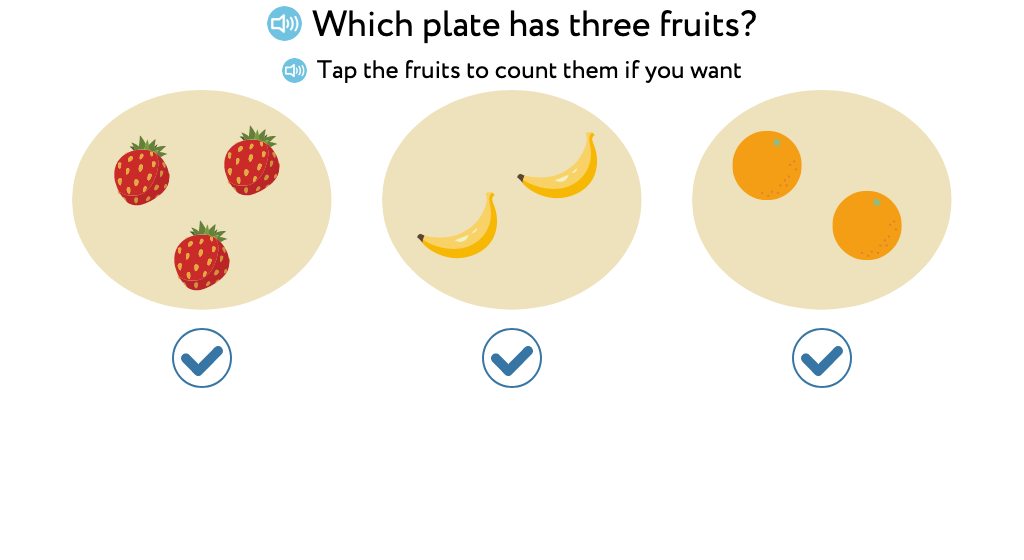#### Counting up to 3 practice

Practice counting 1, 2, and 3. Help Dino feed the goat and the rabbits by counting out 1 step, 2 steps, or 3 steps. The number of steps to take is shown by a numeral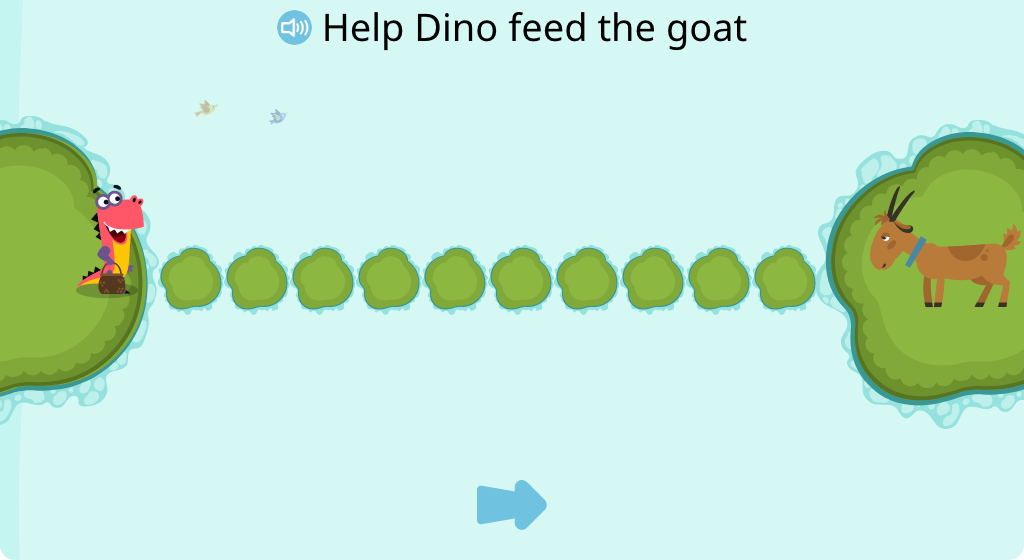### Topic E: Totals of 4 and 5

Students build their ability to count with 1:1 matching and move in a left to right progression. They are introduced to the concept of "a pair" and develop their visual recognition of totals without counting. Students begin to create parts (or groups) for totals of 4 or 5 objects.

#### Count to identify the total of up to 5 identical objects

Practice counting up to 5 identical objects in a row. Click the number that shows the answer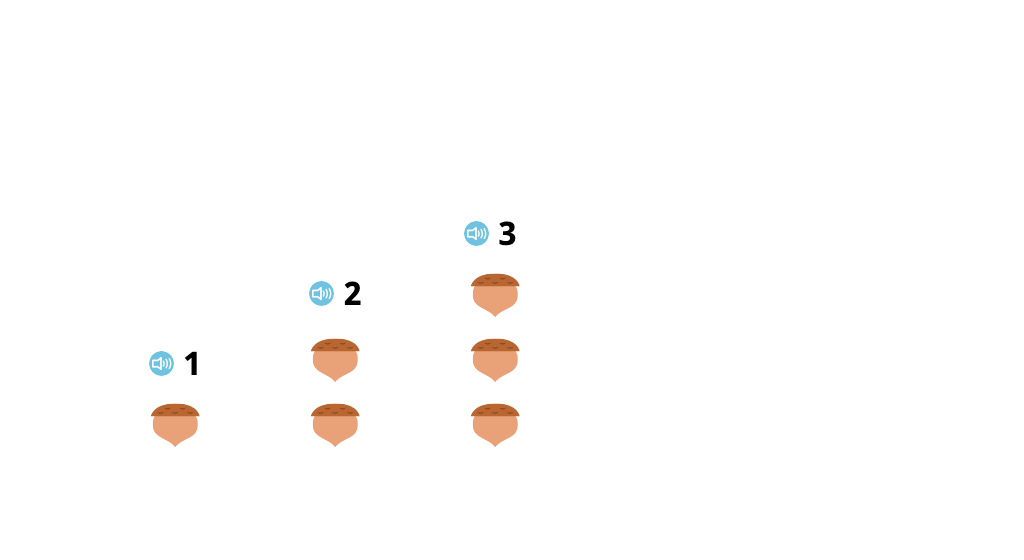#### Number up to 5 objects from left to right

Number up to five objects from left to right by clicking on the numbers in order, up to a given number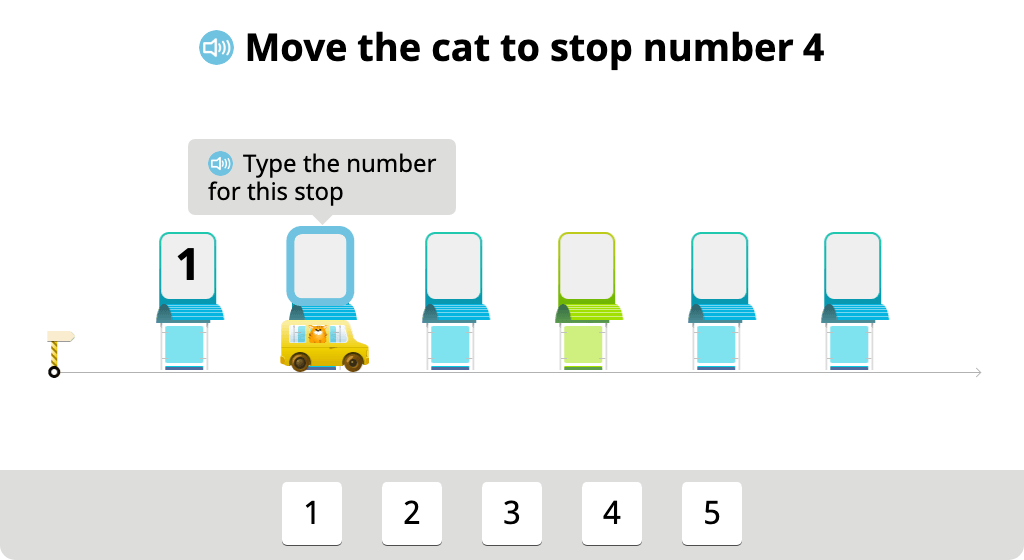#### Identify a set of objects with a given total

Creatures called numberlings are holding stones. Click on the numberlings with a given number of stones to practice identifying a set of objects with a given total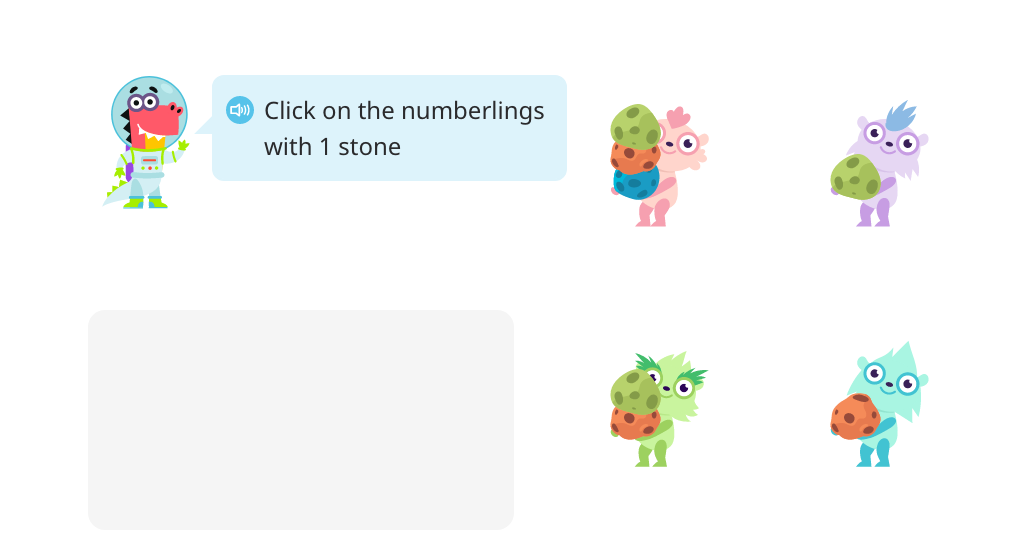#### Separate pairs of objects from a group of 4

Practice grouping objects in pairs by putting two objects in each group. Recognize that a pair means two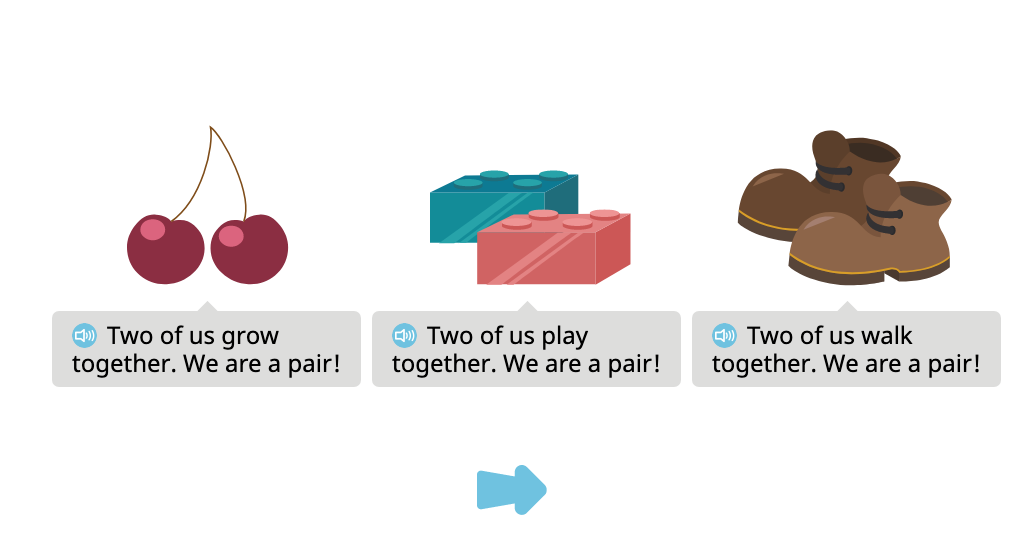#### Separate objects into two pairs and count to reach a total of 4

Practice grouping objects in two pairs and then counting to find the total of four. Click on the correct number to tell how many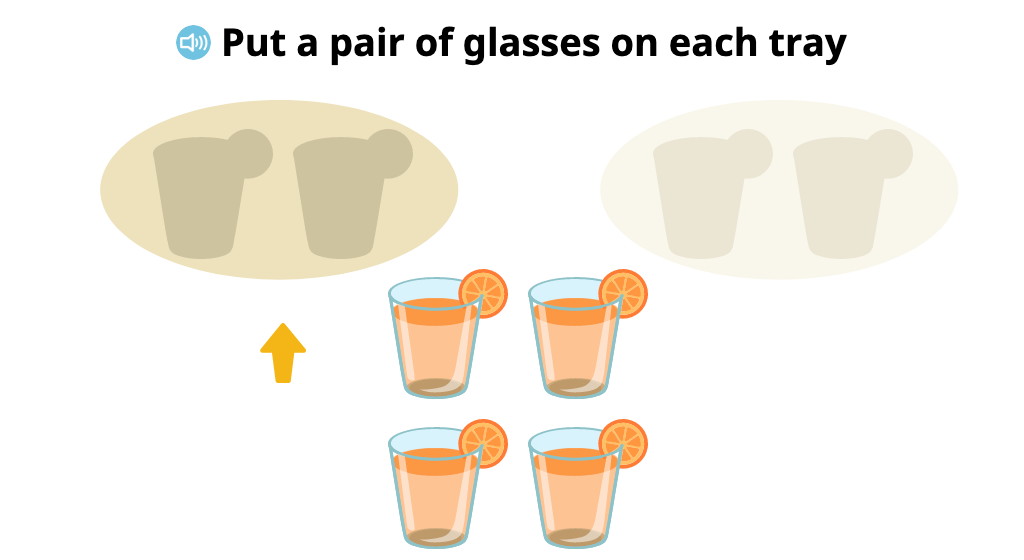#### Identify totals of up to 4 identical objects

Practice subitizing up to four objects. Try to tell how many without counting each object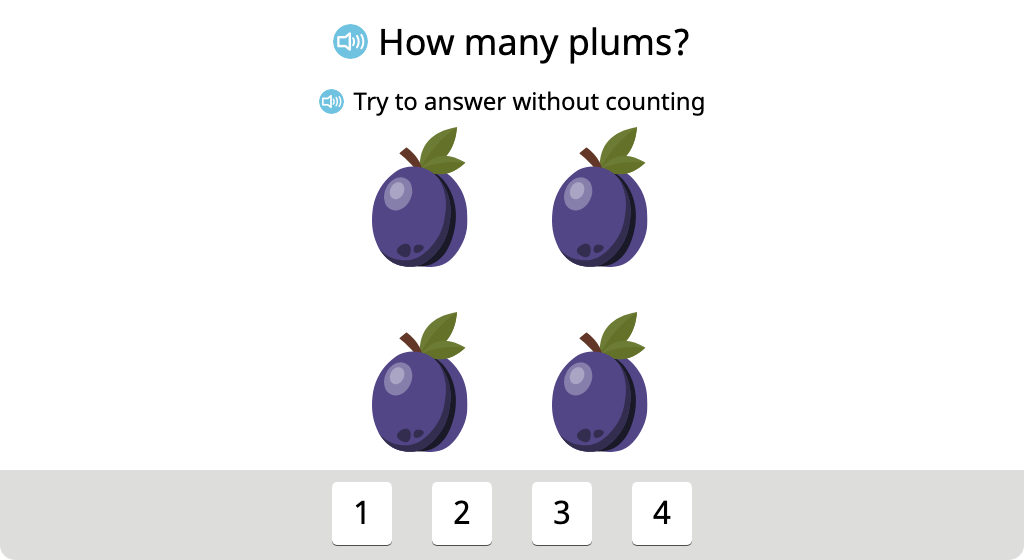#### Split a set of 4 objects into two groups in different ways (Part 1)

Practice splitting 4 objects in two different ways. See that 4 objects can be split into 1 and 3 or 2 and 2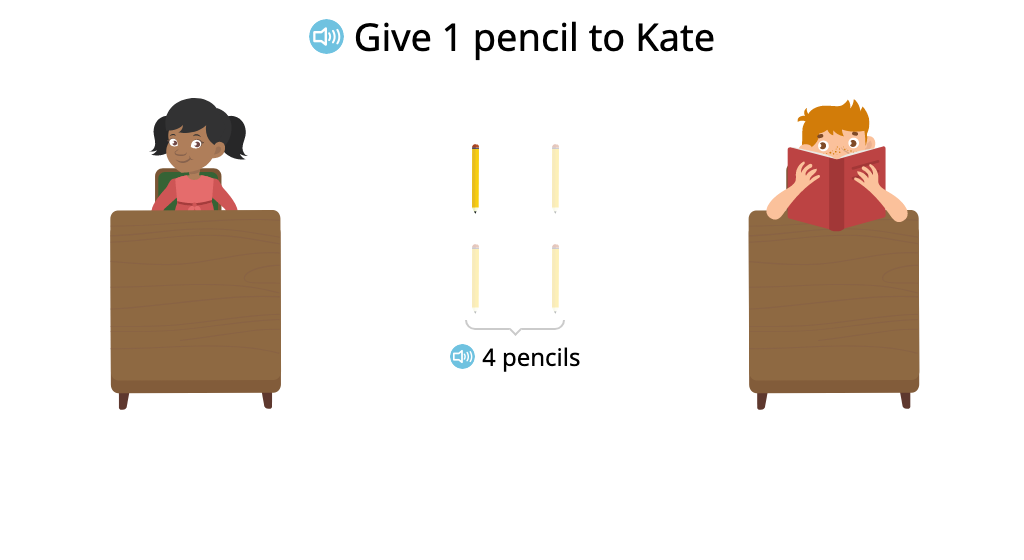#### Split a set of 4 objects into two groups in different ways (Part 2)

Practice splitting 4 objects in two different ways. See that 4 objects can be split into 1 and 3 or 2 and 2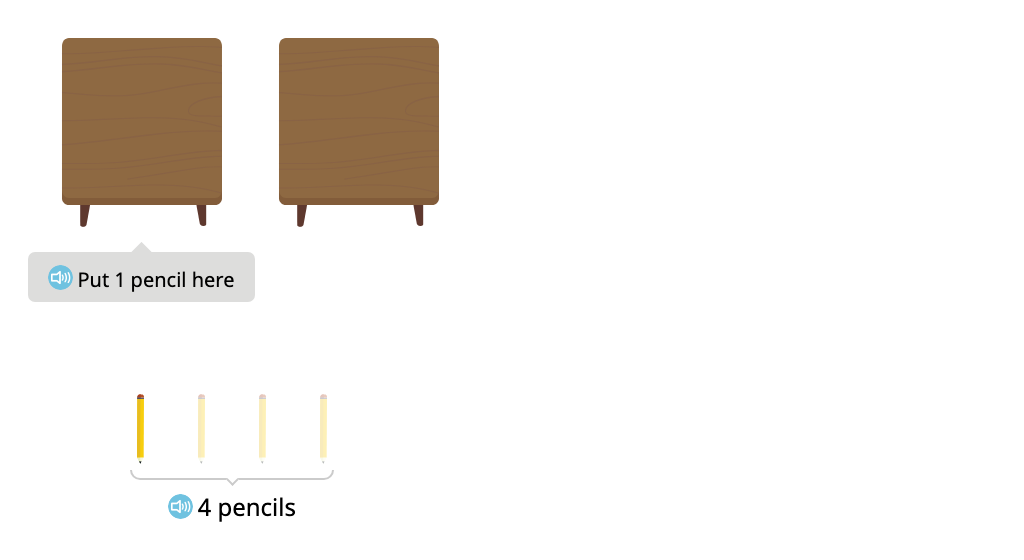#### Split a set of 5 objects into two groups in different ways (Part 1)

Practice splitting 5 objects in two different ways. See that 5 objects can be split into 1 and 4 or 2 and 3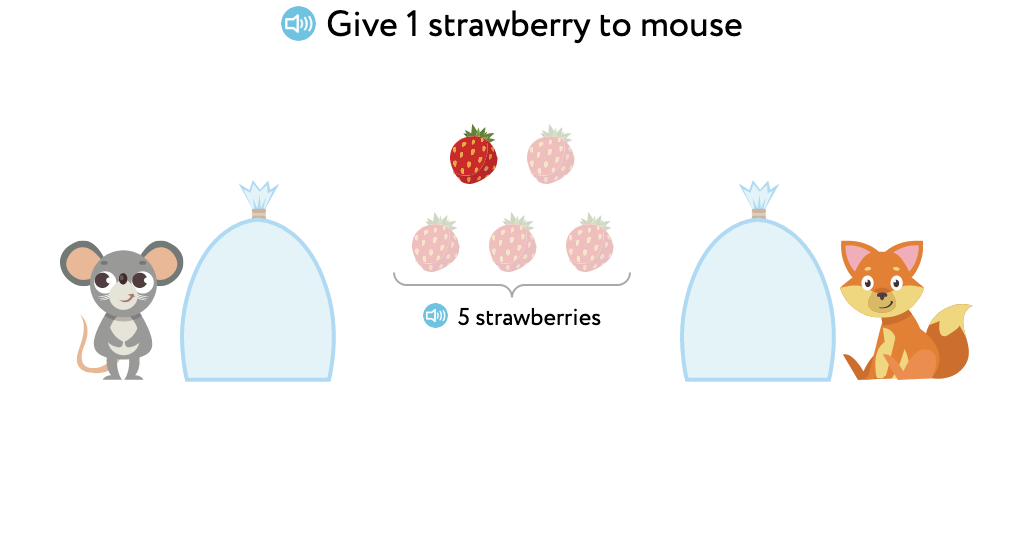#### Split a set of 5 objects into two groups in different ways (Part 2)

Practice splitting 5 objects in two different ways. See that 5 objects can be split into 1 and 4 or 2 and 3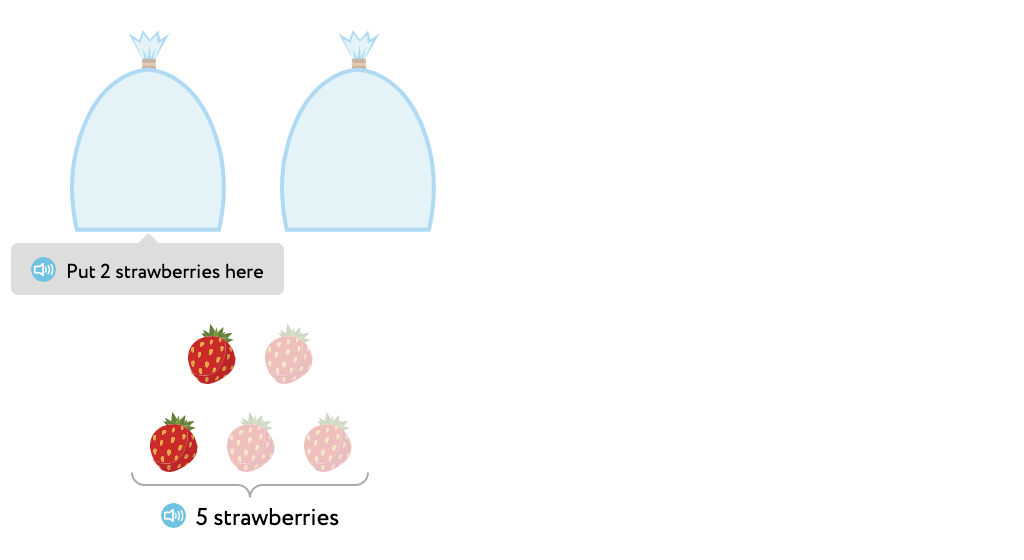### Topic F: Matching 1 Numeral with up to 5 Objects

Students match groups of uniform objects to a total written in digits. They work with both aligned and scattered objects to match objects to a number or match a number to objects. They do not necessarily count each individual object, but are encouraged to "see" the total.

### Topic G: One More with Numbers 1 to 5

Students work with sets of identical objects to determine the total when one more is added. They may or may not count each object as they begin to rely on their ability to visually recognize totals up to five.

### Topic H: Counting 5, 4, 3, 2, 1

Students work with sets of identical objects to determine the total when one is removed. They may or may not count each object as they begin to rely on their ability to visually recognize totals up to five.

## MODULE 2 Shapes

### Topic A: Two-Dimensional Shapes

Students identify circles, squares, triangles, and rectangles of different sizes, colors, and orientation. Students also work with triangles and rectangles with different proportions. They learn the positions above, below, next to, in front of, and behind.

#### Identify circles from among a set of two-dimensional shapes

Practice recognizing circles. Then find and click on the circles in a set of shapes to feed them to the creature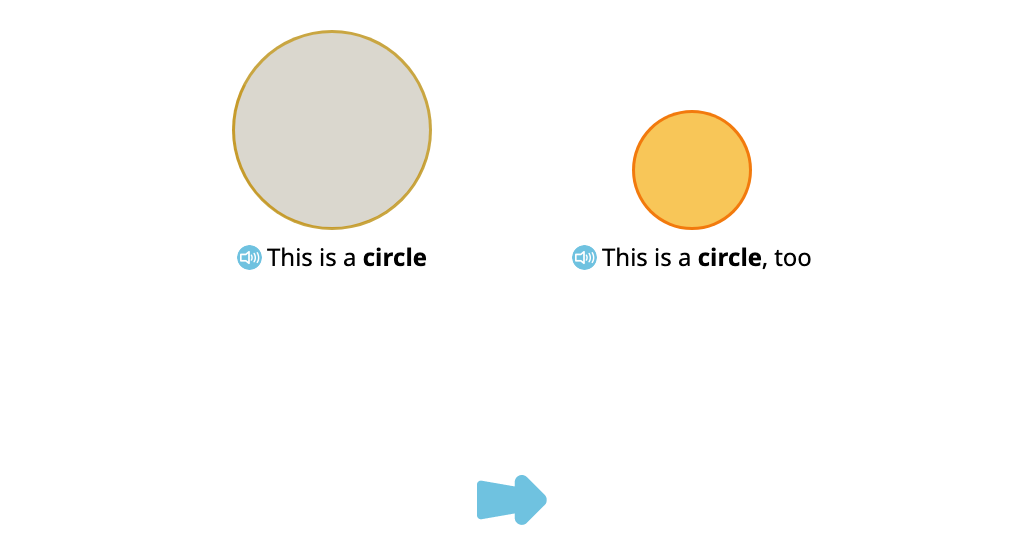#### Identify squares from among a set of two-dimensional shapes

Practice recognizing squares. Then find and click on the squares to feed them to the creature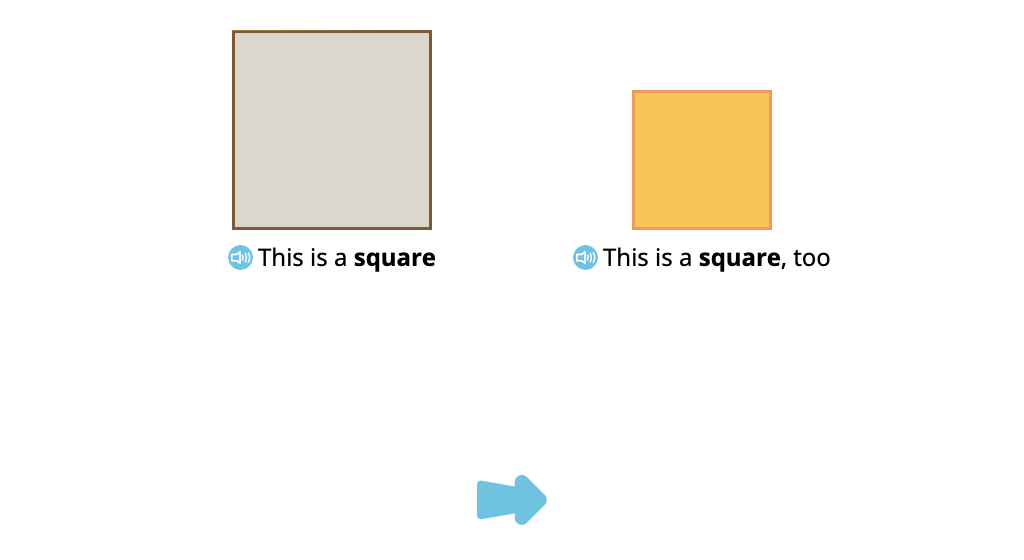#### Identify triangles from among a set of two-dimensional shapes

Practice recognizing triangles. Then find and click on the triangles to feed them to the creature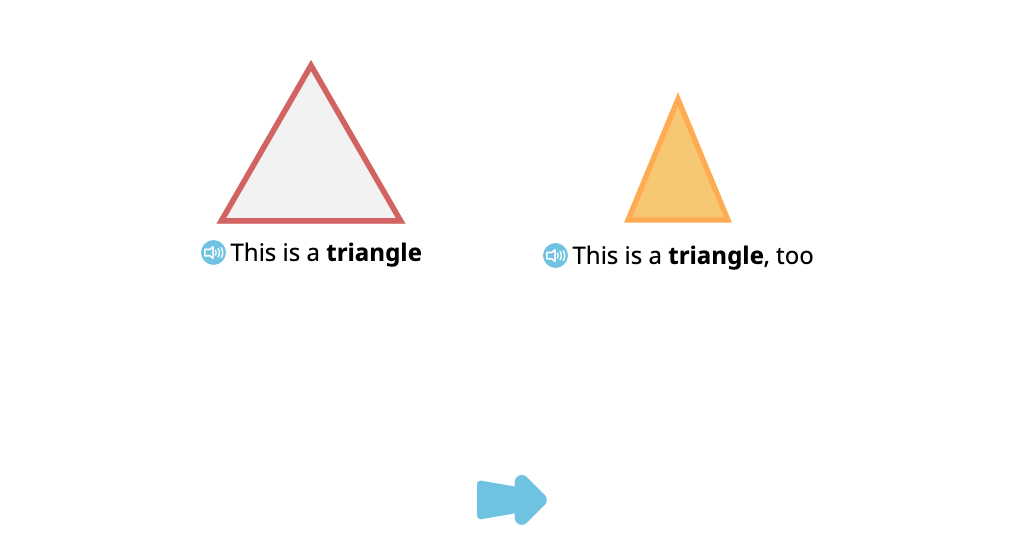#### Identify rectangles from among a set of two-dimensional shapes

Practice recognizing rectangles. Then find and click on the rectangles to feed them to the creature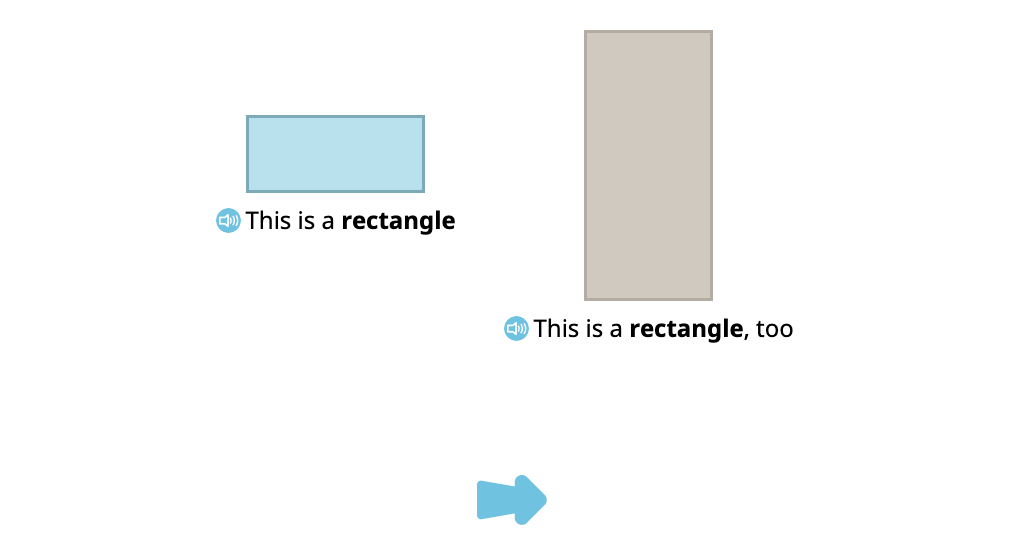#### Identify the position above or below a given object (Part 1)

Practice the positional words "above" and "below." Help guide a spaceship through space by telling it to go above or below an asteroid#### Identify the position above or below a given object (Part 2)

Practice the positional words "above" and "below" by tapping the space above or below the birds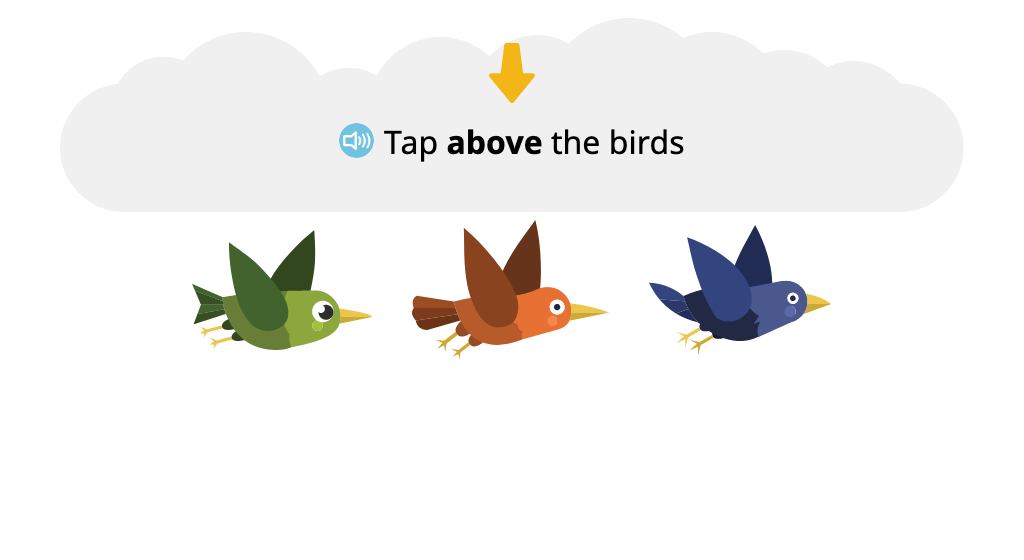#### Move objects up or down as instructed

Practice the words "up" and "down" by dragging handles up or down to reveal a space scene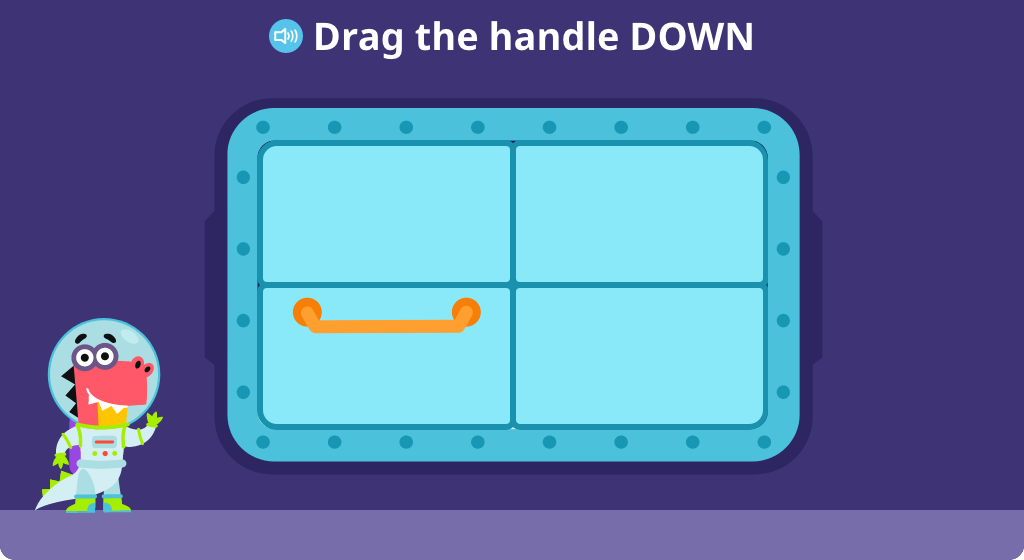#### Position a shape above or below another shape as instructed

Practice the words "above" and "below." Listen to the directions telling where to put the given shapes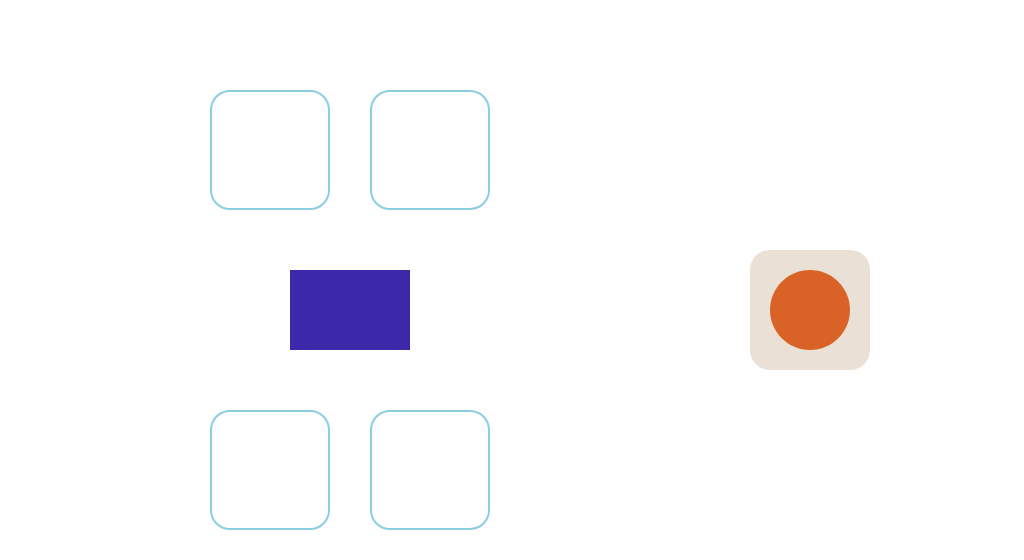#### Position an object next to another object as instructed

Practice the meaning of the positional phrase "next to" by positioning objects next to other objects#### Identify an object based on the position near or between

Practice the meaning of the positional words "near" and "between" by locating a hiding creature based on a spoken clue#### Position an object in front of or behind another object as instructed

Practice the positional terms "in front of" and "behind" by placing objects in front of or behind other objects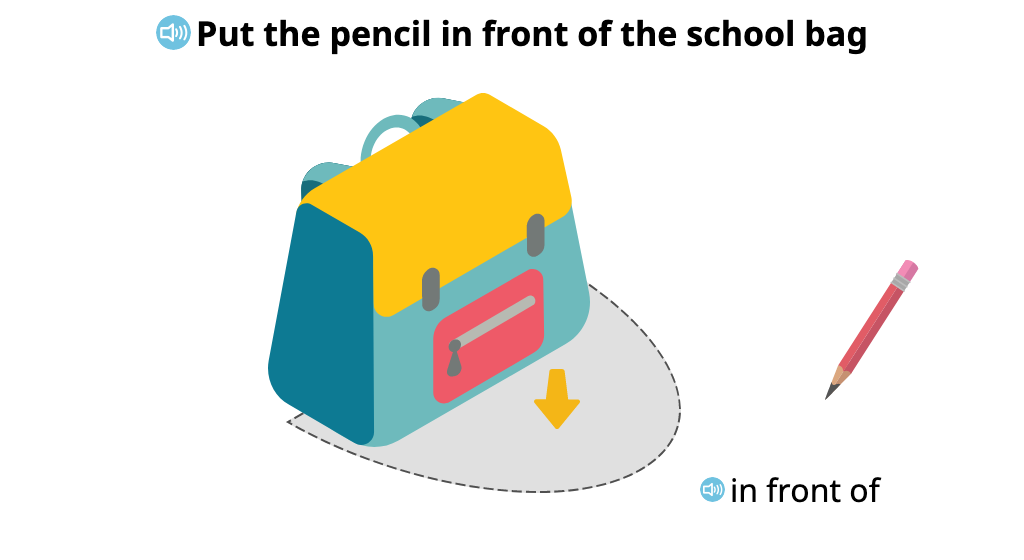## MODULE 3 Counting to 10

### Topic A: How Many Questions with up to 7 Objects

Students continue developing their ability to count with 1:1 matching, moving in a left to right progression as well as from top to bottom to count objects in rows. They continue to develop their visual recognition of totals without counting.

#### Recognize that one more than 5 is 6

Learn that one more than 5 is 6. Count a row 5 objects, then see one more object added to the row. Learn that the next number after 5 is 6 as a 6 tile is placed beside the 5 tile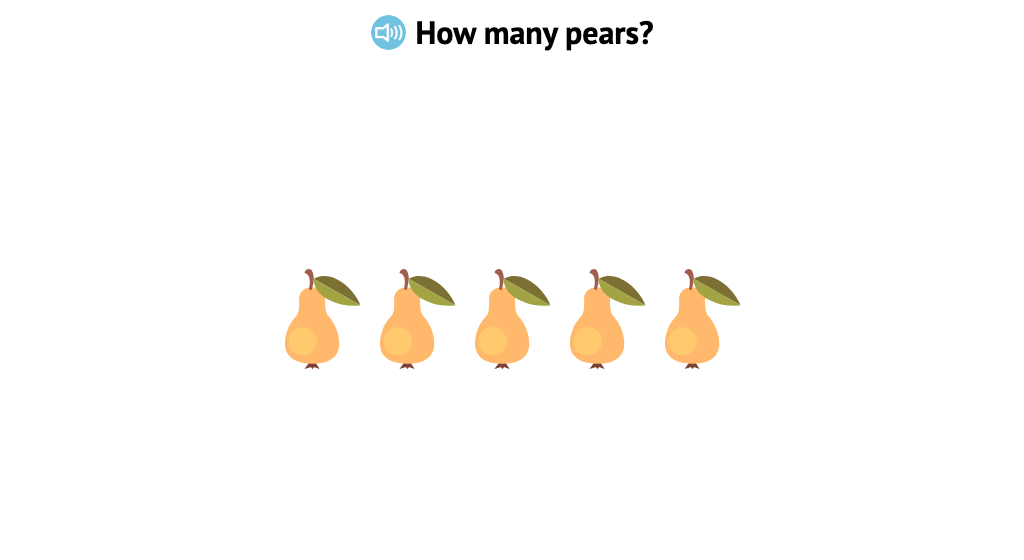#### Count 6 similar aligned objects from left to right

Count up to 6 similar objects that are in a row. Practice one-to-one correspondence by clicking on each object as you count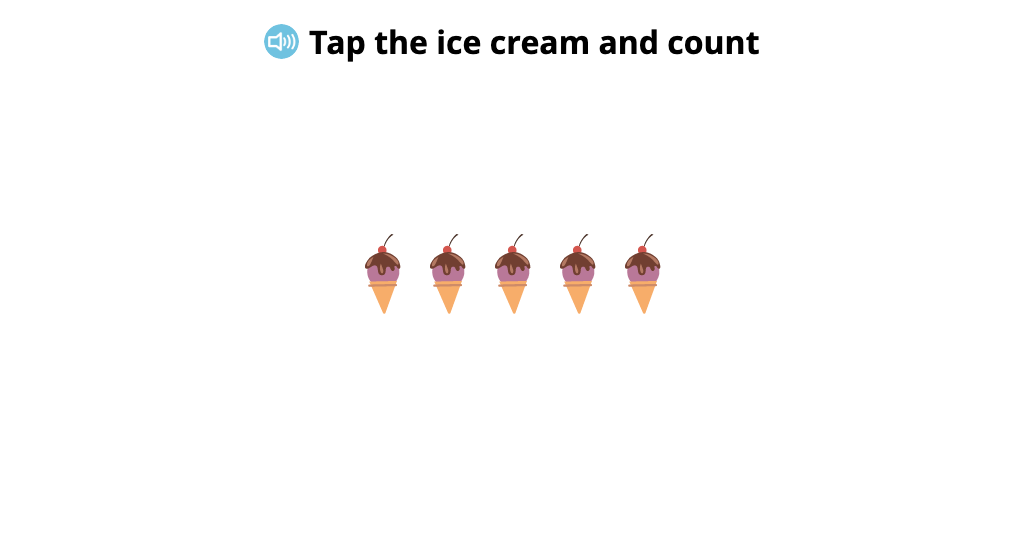#### Tap objects to count the total to 6

Count 6 objects that are not in a row, and click on the number that tells how many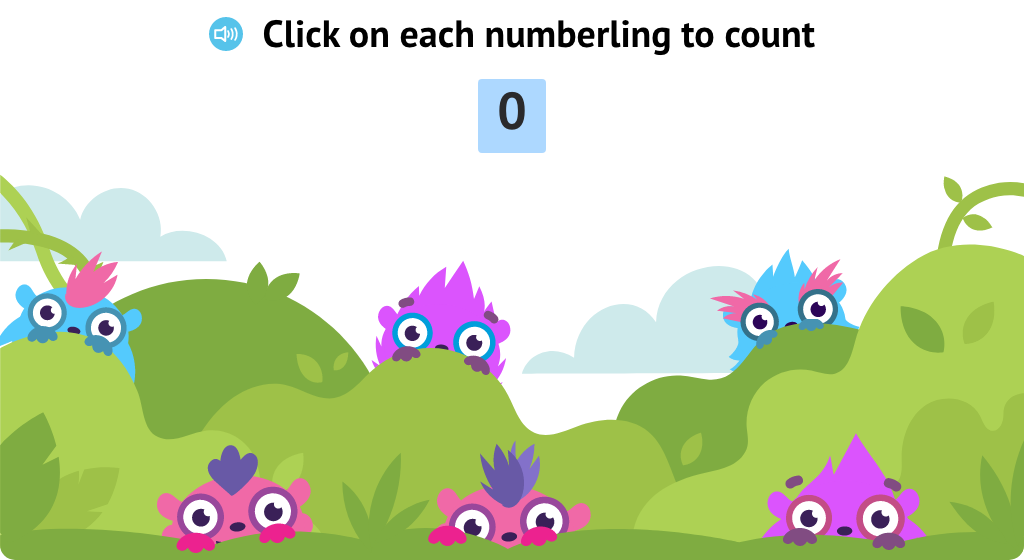#### Identify totals of up to 6 identical objects

Count up to 6 objects, then click on the number that tells how many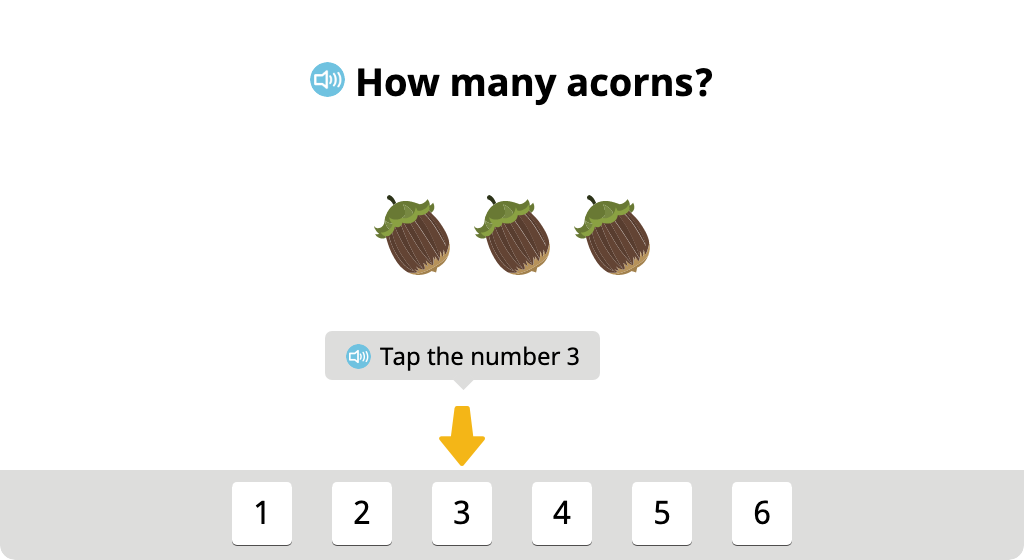#### Recognize that one more than 6 is 7

Learn that one more than 6 is 7. Count a row 6 objects, then see one more object added to the row. Learn that the next number after 6 is 7 as a 7 tile is placed beside the 6 tile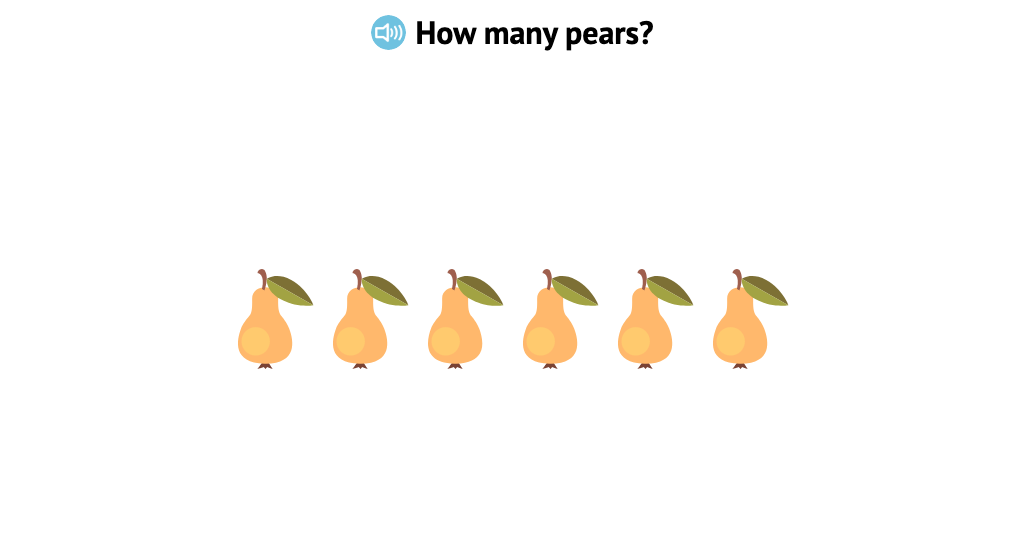#### Count 7 similar aligned objects from left to right

Count up to 7 similar objects that are in a row. Practice one-to-one correspondence by clicking on each object as you count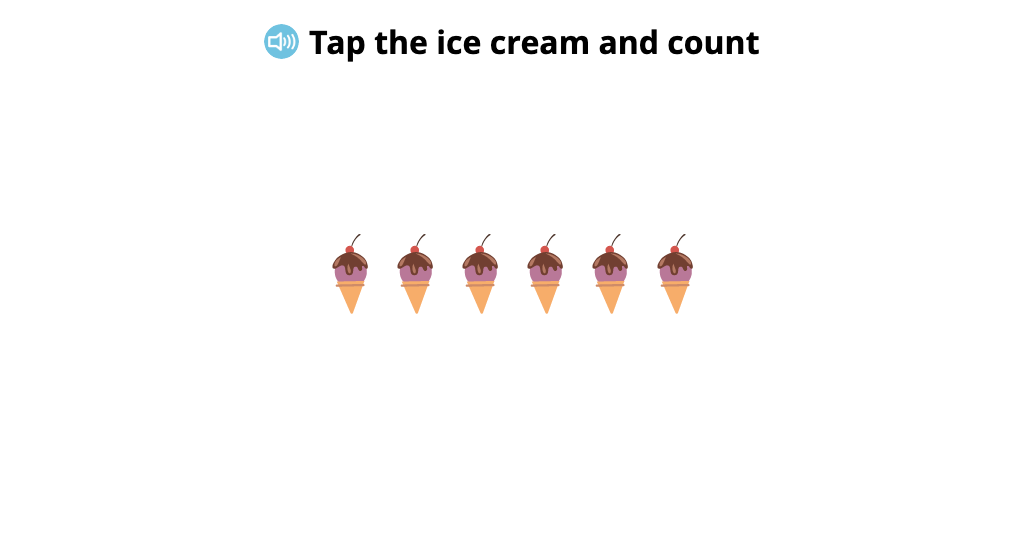#### Tap objects to count the total to 7

Count 7 objects that are not in a row, and click on the number that tells how many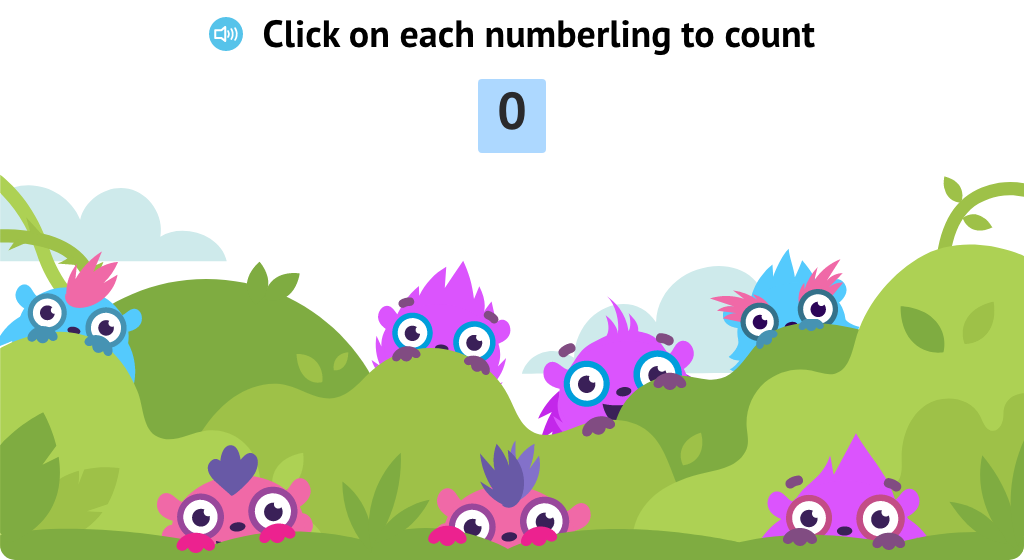#### Identify totals of up to 7 identical objects

Count up to 7 similar objects that are in a row. Click on the number that tells how many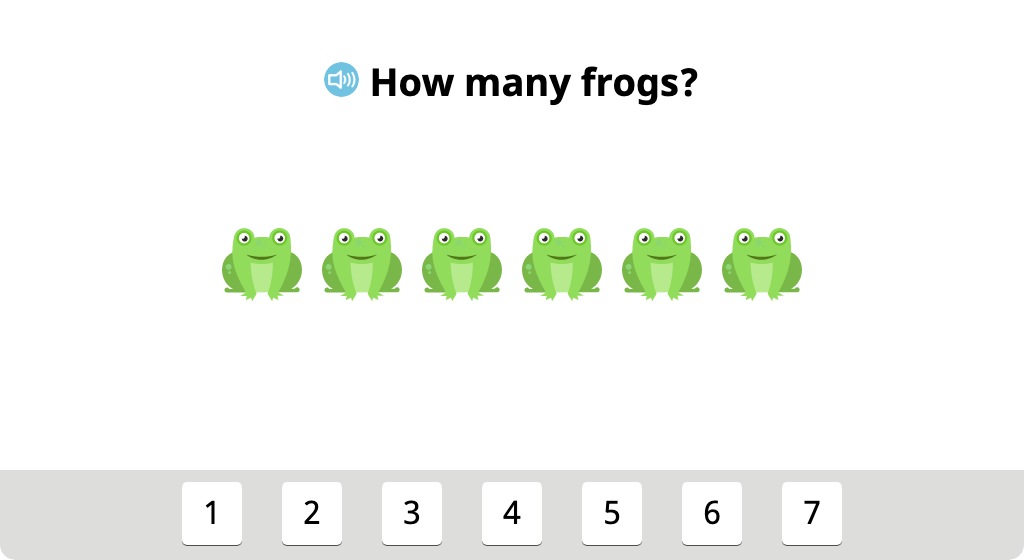#### Align groups of identical objects and identify the total

Learn that it is easier to count objects when they are in a row. Line up 6 or 7 objects in a row, then count. Click on the number that tells how many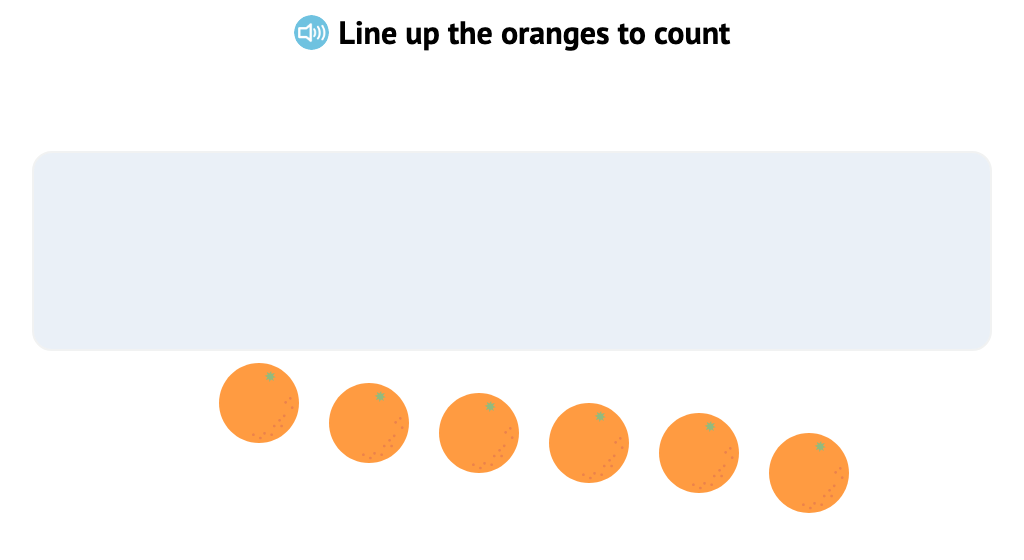#### Count identical objects in two rows from left to right, top to bottom

Practice counting up to 6 objects that are placed in two rows by counting left to right and top to bottom### Topic B: Matching One Numeral with up to 7 Objects

Students work with groups of identical objects to split totals in different ways (a precursor to learning fact families). They count objects in different alignments, including circular, two rows, and vertical column. In the final exercise, they see that when objects are rearranged, the total remains the same.

#### Combine and split groups of 6 identical objects and determine totals

Count how many pencils a girl has, then count how many pencils a boy has. Then, put all of the pencils together and count how many there are altogether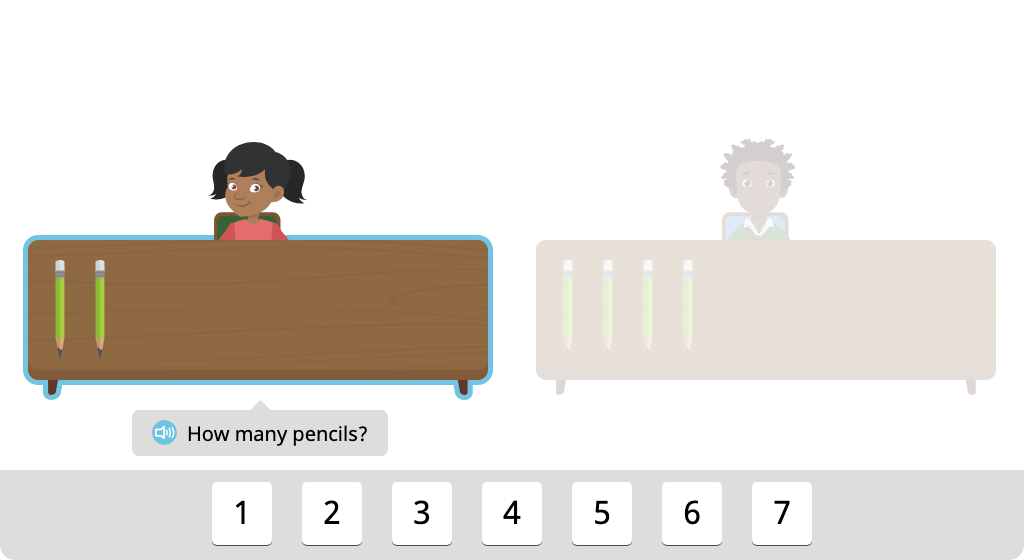#### Split a group of 6 identical objects into two groups in different ways

Split 6 flowers into two groups in different ways. First, split the flowers into two groups of 3. Then split the flowers into a group of 1 and a group of 5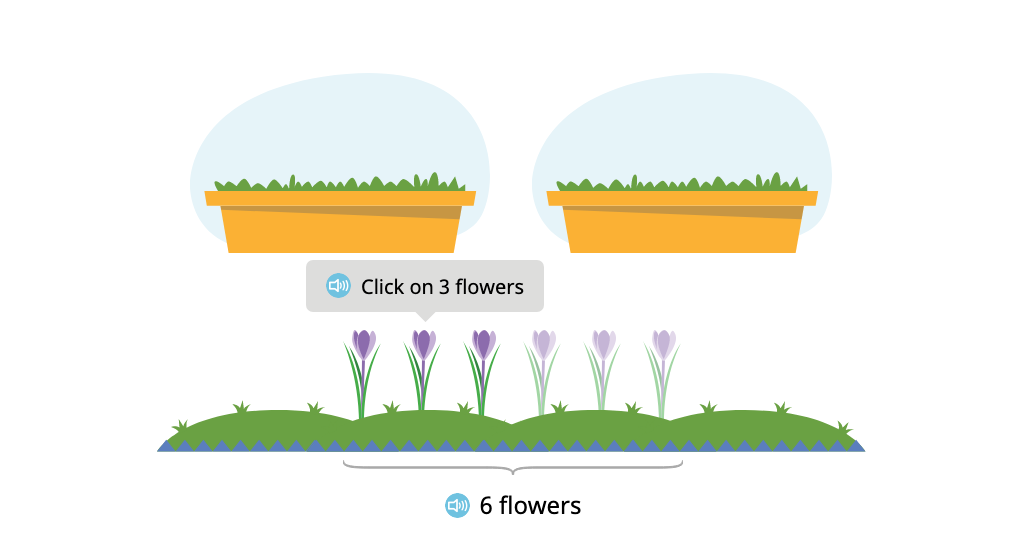#### Combine and split groups of 7 identical objects and determine totals

Count how many pencils a girl has, then count how many pencils a boy has. Then, put all of the pencils together and count how many there are altogether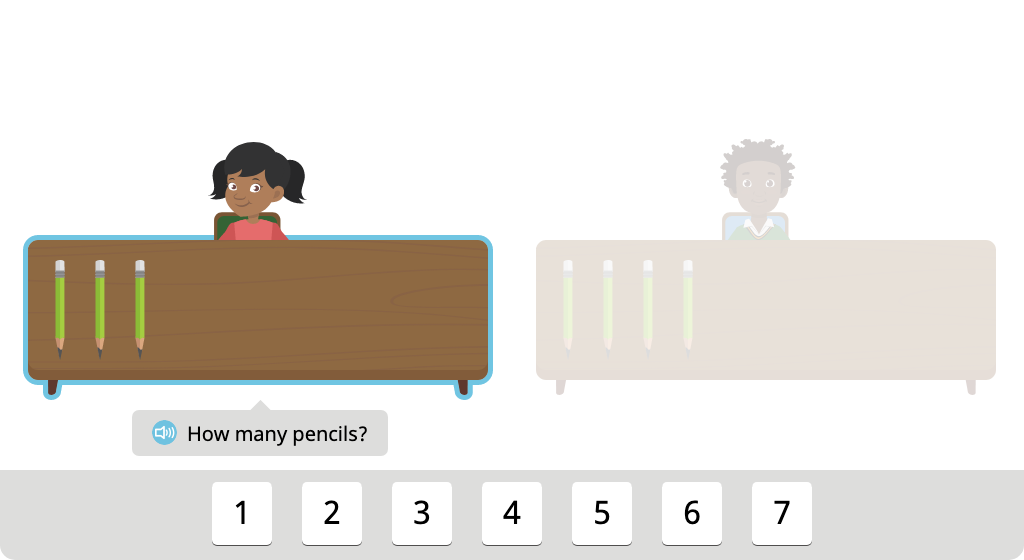#### Split a group of 7 identical objects into two groups in different ways

Split 7 flowers into two groups in different ways. First, split the flowers into a group of 3 and a group of 4. Then split the flowers into a group of 1 and a group of 6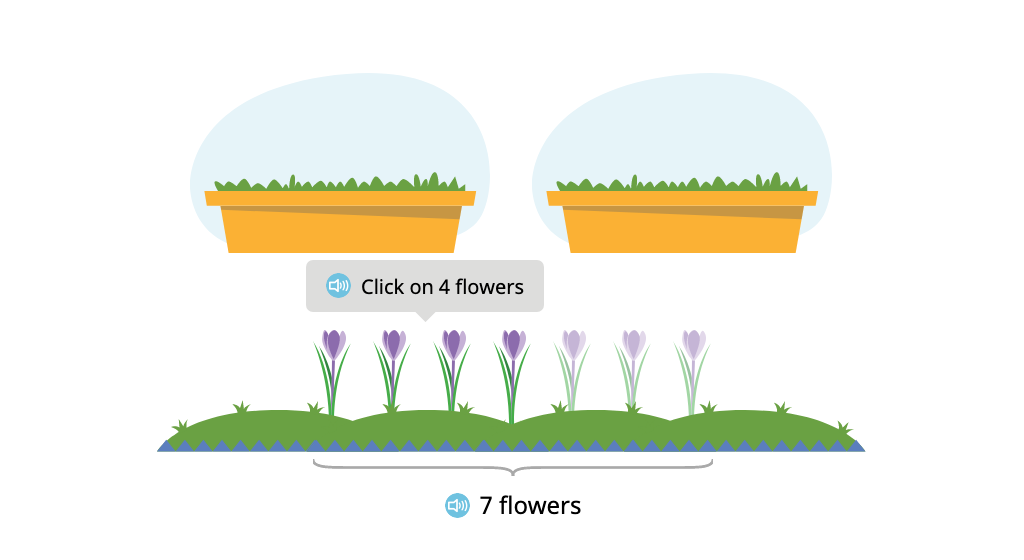#### Distribute and count 7 identical objects aligned in a circular arrangement

Distribute 7 balls to 7 children who are standing in a circle. Then count the balls and click on the number to tell how many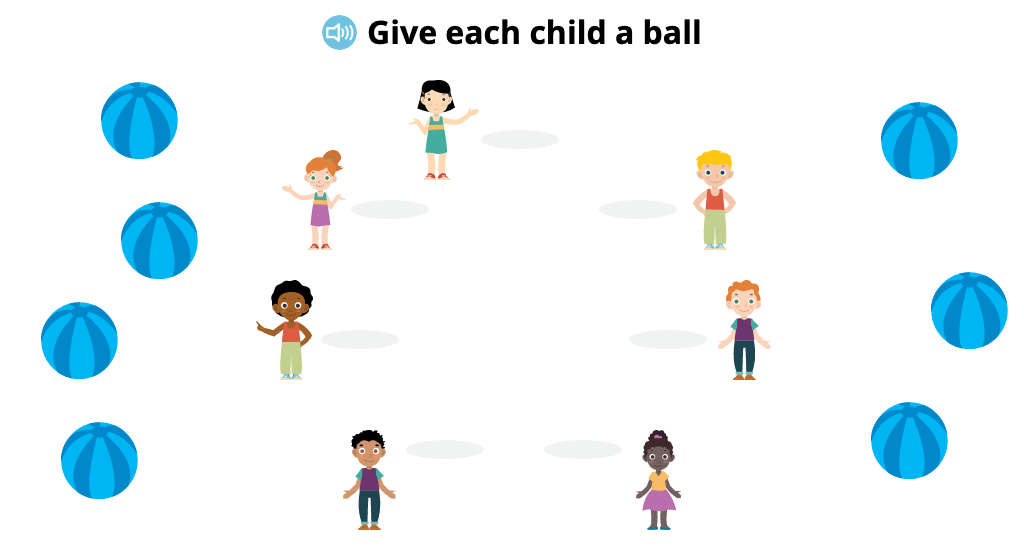#### Distribute and count 6 identical objects aligned in two rows

Practice one-to-one correspondence. Distribute 6 bowls of food to 6 pets who are sitting in two rows. Then count the bowls to tell how many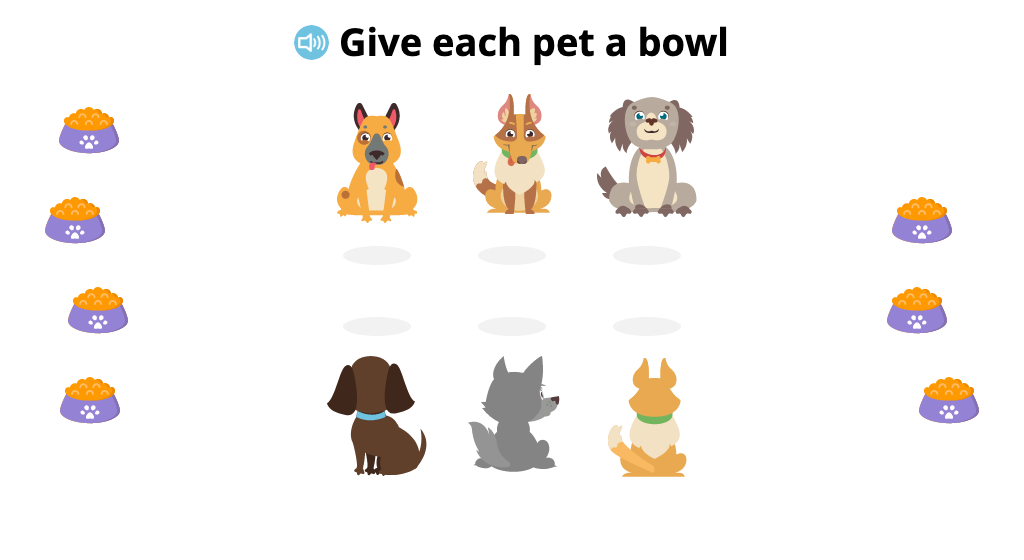#### Distribute and count 5 or 6 identical objects aligned in a column

Practice counting objects that are in a column. Sew 5 or 6 buttons on a jacket. Then count the buttons and click on the number to tell how many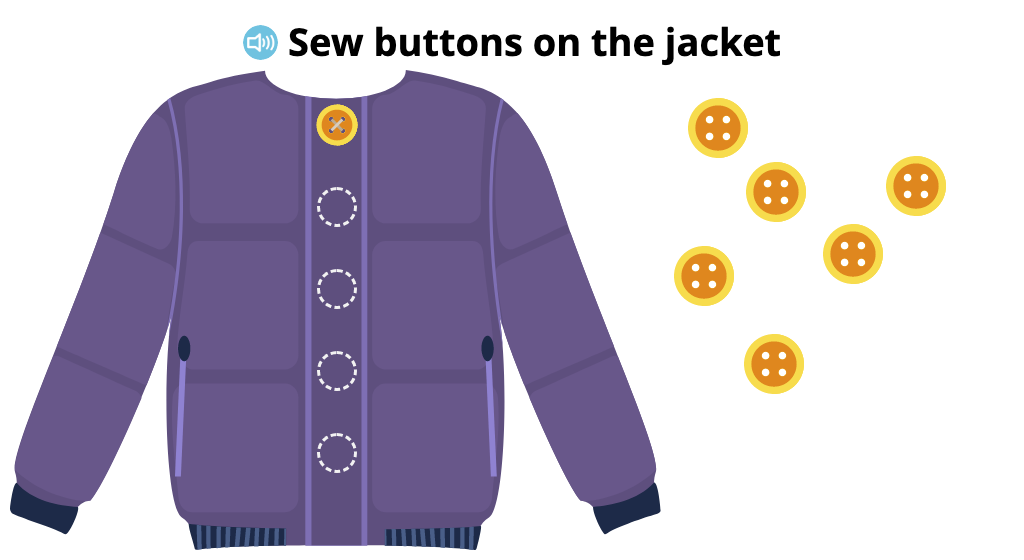### Topic C: How Many Questions with up to 8 Objects

Students continue developing their ability to count with 1:1 matching, moving in a left to right progression as well as from top to bottom to count objects in rows.

#### Recognize that one more than 7 is 8

Learn that one more than 7 is 8. Count a row 7 objects, then see one more object added to the row. Learn that the next number after 7 is 8 as an 8 tile is placed beside the 7 tile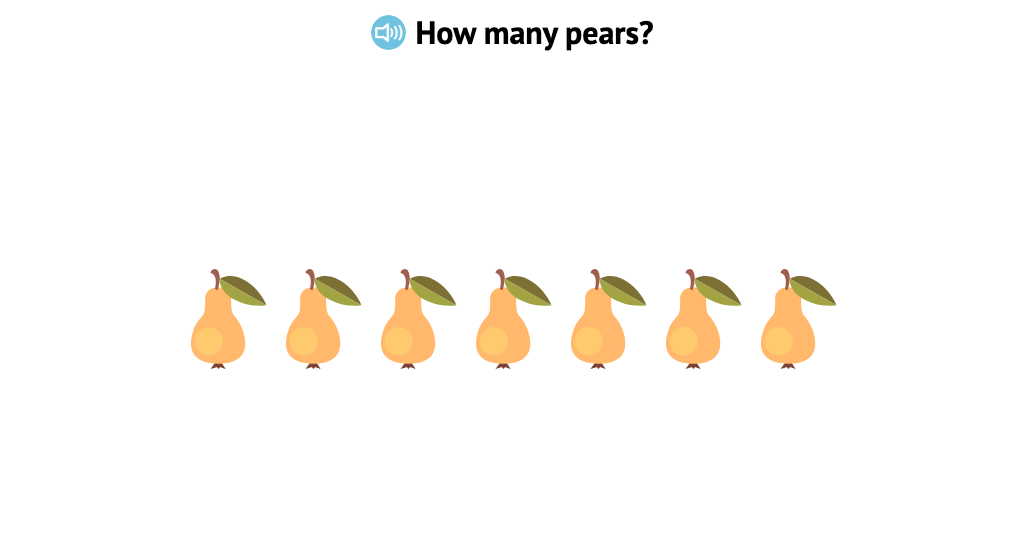#### Count 8 similar aligned objects from left to right

Count up to 8 similar objects that are in a row. Practice one-to-one correspondence by clicking on each object as you count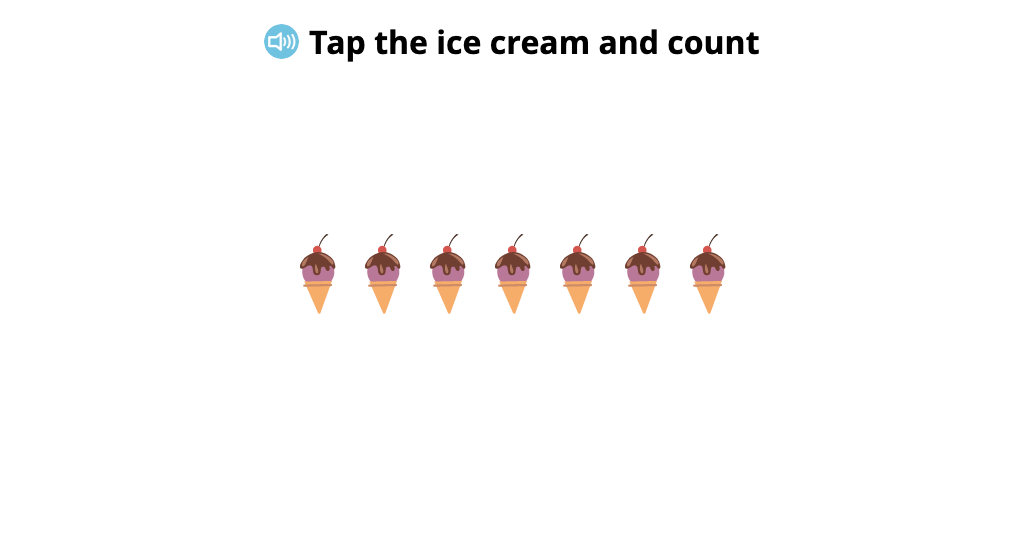#### Identify totals of up to 8 identical objects

Practice counting. Count up to 8 identical objects that are in a row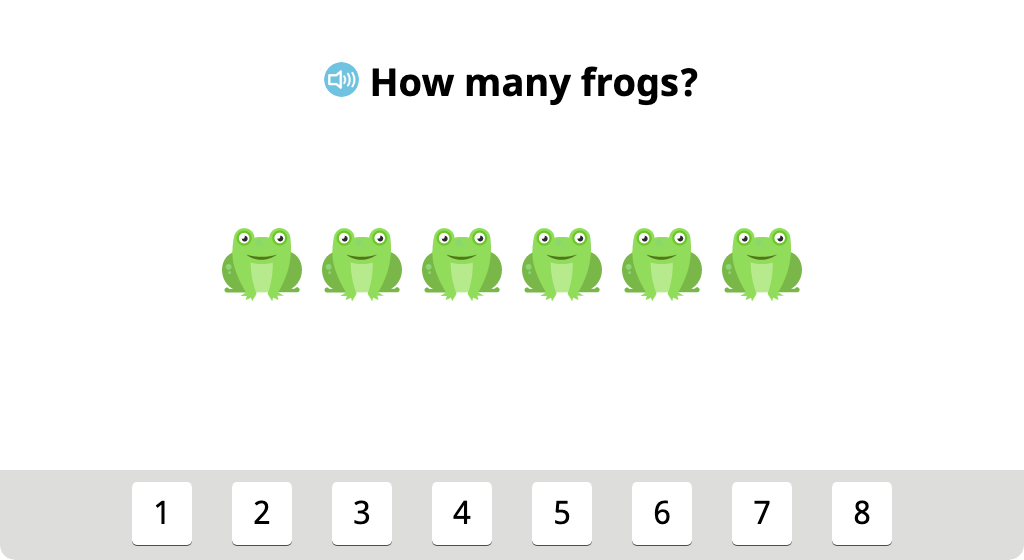#### Align groups of identical objects and identify the total

See that it is easier to count if you line up the objects in a row first. Align up to 8 objects in a box, then count them and click on the number that tells how many there are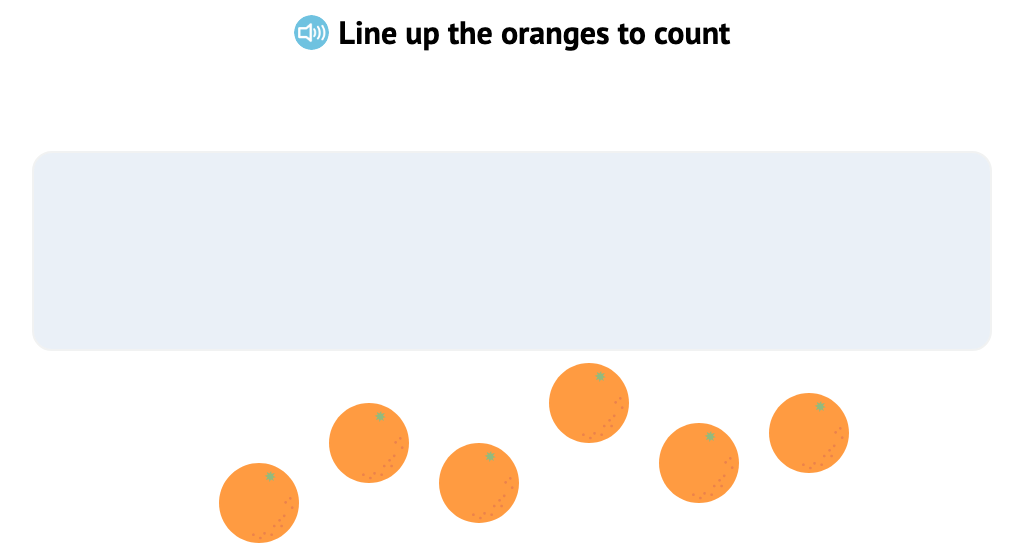#### Count identical objects in two rows from left to right, top to bottom

Practice counting up to 8 objects that are placed in two rows by counting left to right and top to bottom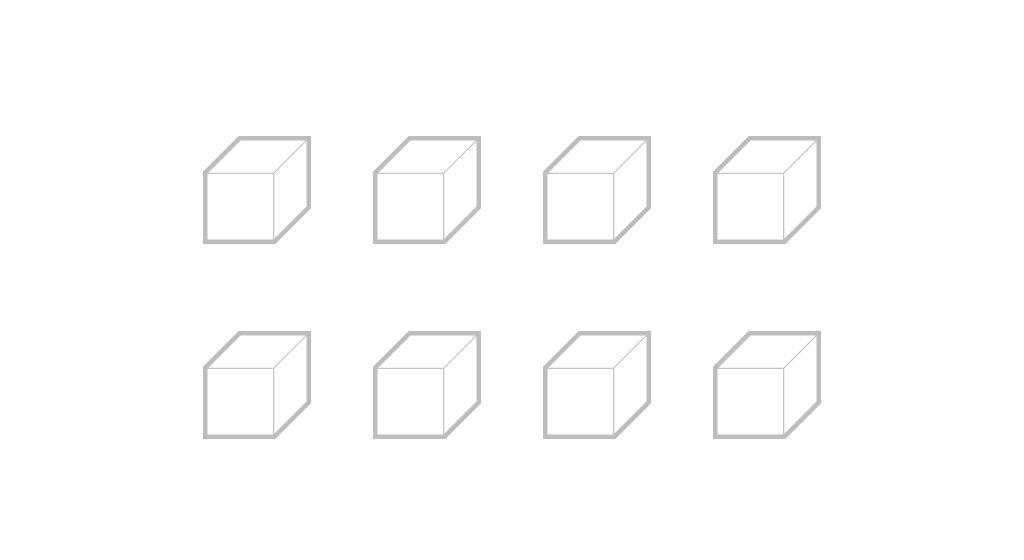### Topic D: Matching One Numeral with up to 8 Objects

Students match groups of uniform objects to a total written in digits. They work with both aligned and scattered objects to match objects to a number or match a number to objects. Students begin to create parts (or groups) for totals of up to eight objects.

#### Identify the total of 8 identical objects aligned in two groups

Count how many pencils a girl has, then count how many pencils a boy has. Then, put all of the pencils together and count how many there are altogether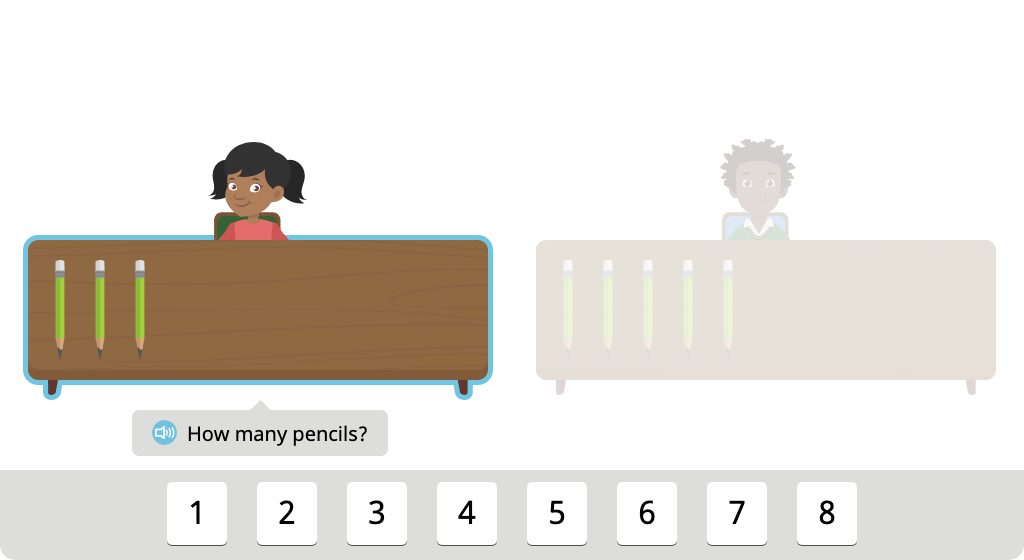#### Split a set of 8 objects into two groups in different ways

Split 8 flowers into two groups in different ways. First, split the flowers into two groups of 4. Then split the flowers into a group of 2 and a group of 6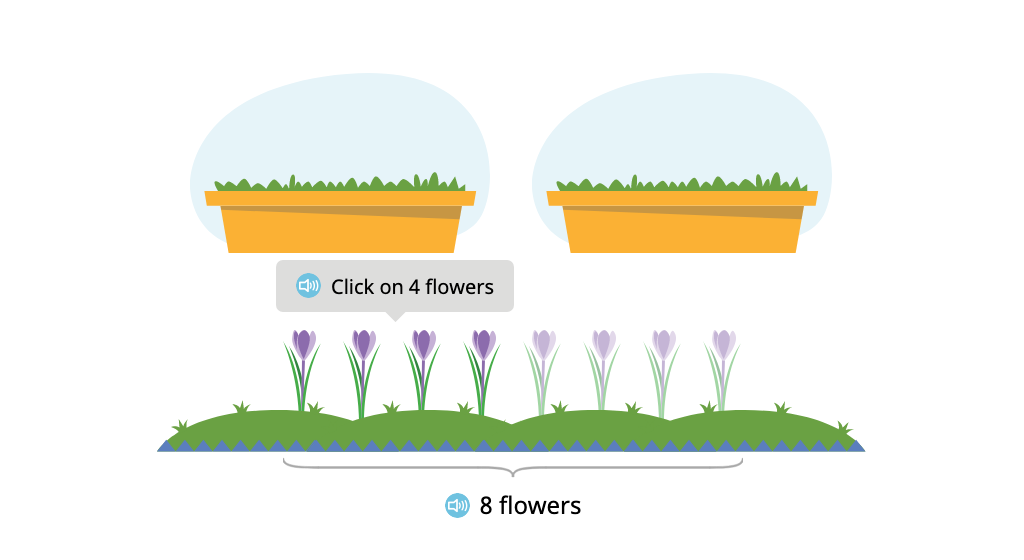#### Identify the total of 8 identical objects aligned in a circle

Distribute 7 balls to 7 children who are standing in a circle. Then count the balls and click on the number to tell how many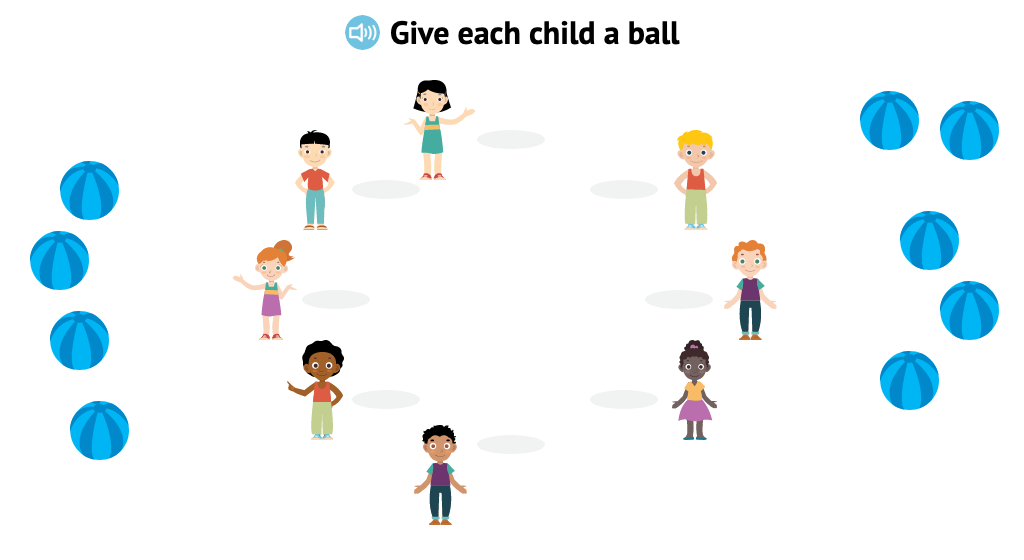#### Identify the total of 8 identical objects aligned in two rows

Distribute bowls of food to 8 dogs who are sitting in two rows. Then count the bowls to tell how many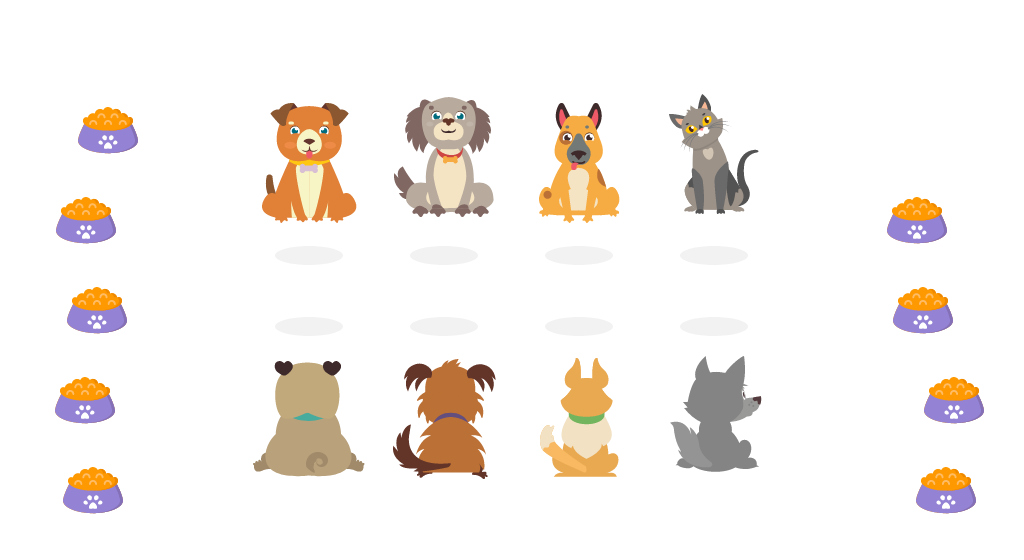#### Identify the total of 7 identical objects aligned vertically

Practice counting objects that are in a column. Sew buttons on a jacket. Then count the buttons and click on the number to tell how many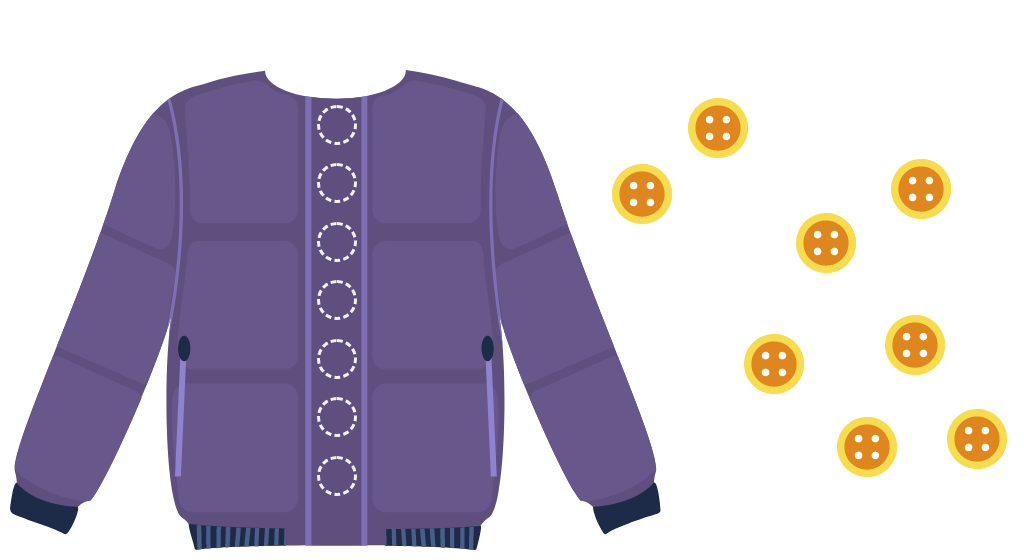### Topic E: How Many Questions with 0 up to 9 Objects

Students are introduced to the concept of 0. They continue developing their ability to count with 1:1 matching, moving in a left to right progression as well as from top to bottom to count objects in rows.

#### Recognize that 0 is an amount that is one less than 1

Learn the meaning of zero. Find one less than 3, one less than 2, and one less than 1. See that the 0 tile goes to the left of 1 in the row of numbers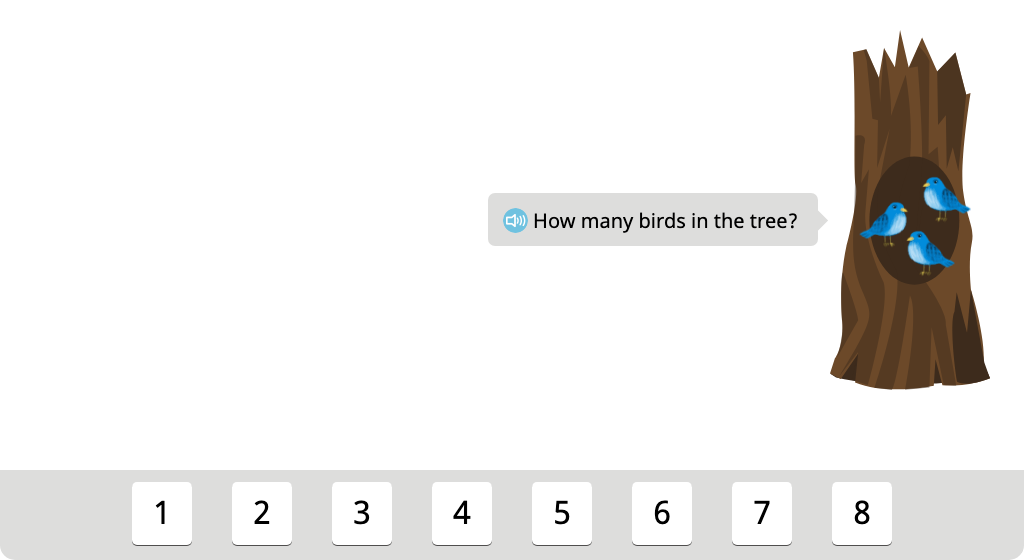#### Determine which set of identical objects has a total of 0

Practice with the concept of zero. Look at three plates and choose the plate that has 0 pieces of fruit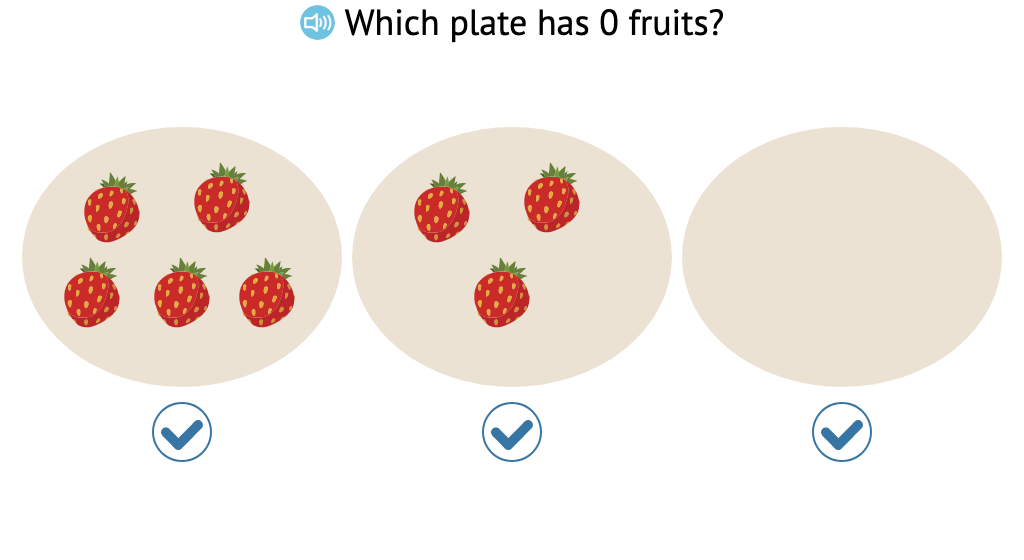#### Determine totals of identical objects, including 0

Practice with the concept of zero. Look at the number of pencils that two children have on their desks. One of the children has 0 pencils. The other child has some pencils. Click on the numbers to tell how many pencils each child has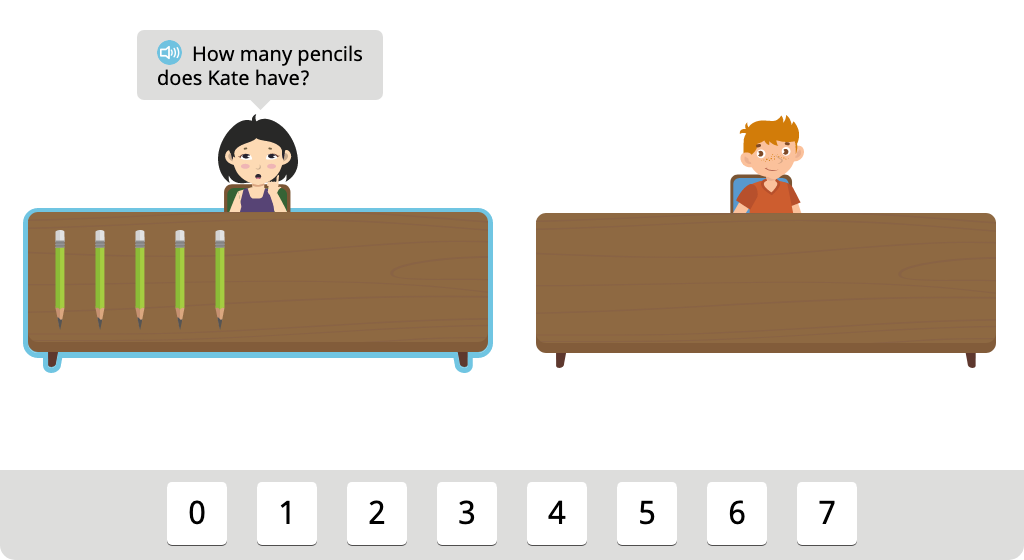#### Recognize that one more than 8 is 9

Learn that one more than 8 is 9. Count a row 8 objects, then see one more object added to the row. Learn that the next number after 8 is 9 as a 9 tile is placed beside the 8 tile#### Count 9 similar aligned objects from left to right

Count up to 9 similar objects that are in a row. Practice one-to-one correspondence by clicking on each object as you count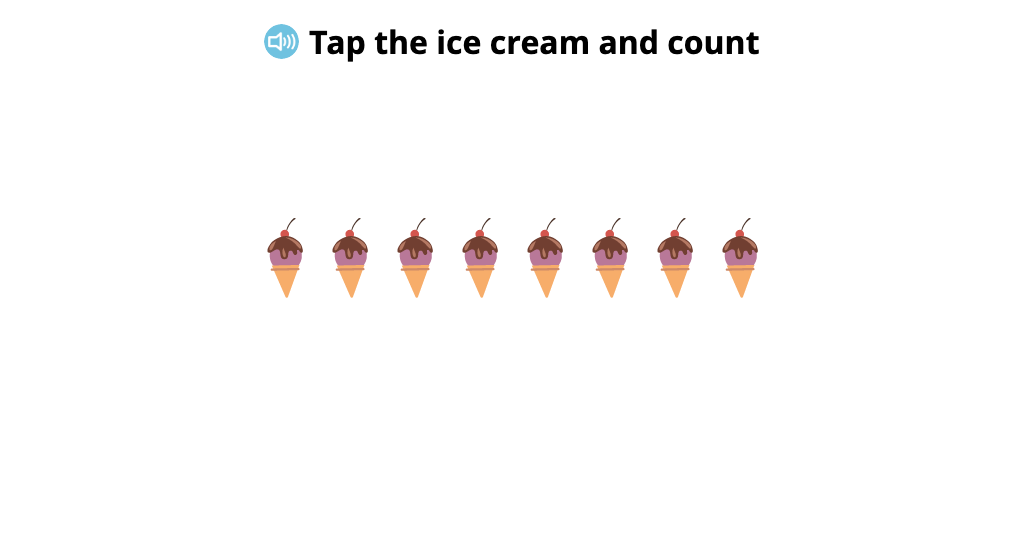#### Identify totals of up to 9 identical objects

Practice counting. Count up to 9 identical objects that are in a row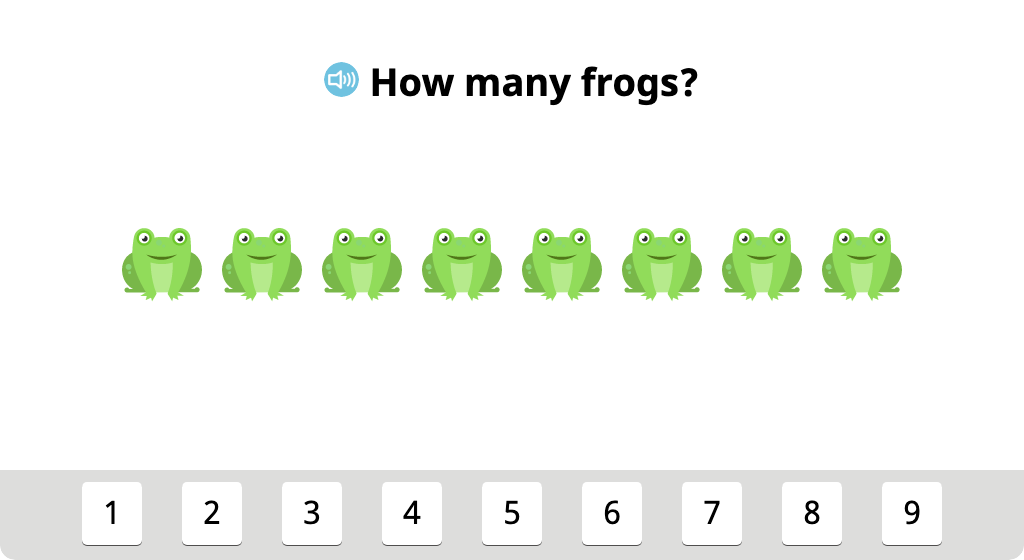#### Align groups of identical objects and identify the total

See that it is easier to count if you line up the objects in a row first. Align up to 9 objects in a box, then count them and click on the number that tells how many there are### Topic F: Matching One Numeral with 0 up to 9 Objects

Students work with groups of identical objects to split totals in different ways (a precursor to learning fact families). They count objects in different alignments, including circular and rows. In the final exercise, they see that when objects are rearranged, the total remains the same.

### Topic G: How Many Questions with up to 10 Objects

Students continue developing their ability to count with 1:1 matching, moving in a left to right progression as well as from top to bottom to count objects in rows.

#### Recognize that one more than 9 is 10

Learn that one more than 9 is 10. Count a row 9 objects, then see one more object added to the row. Learn that the next number after 9 is 10 as a 10 tile is placed beside the 9 tile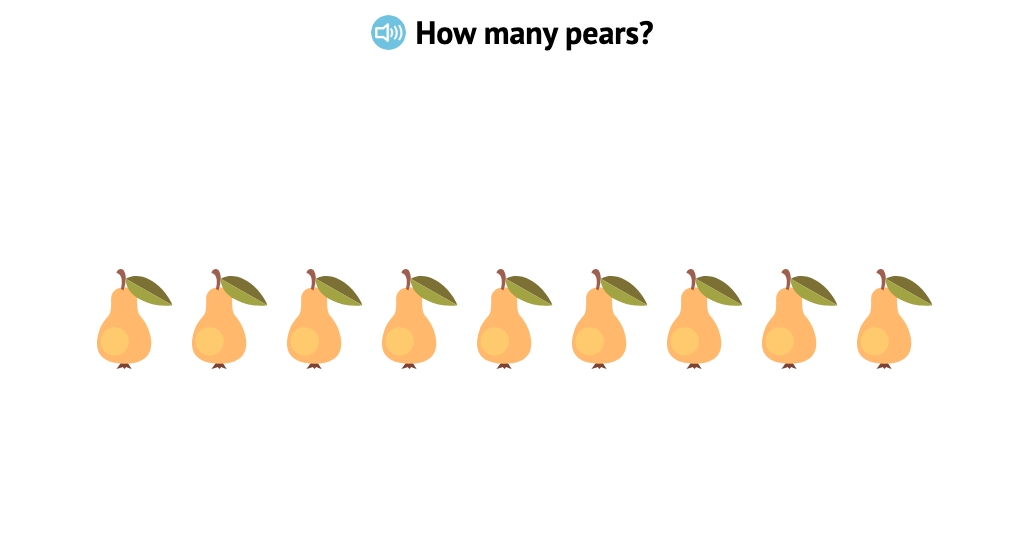#### Count 10 similar aligned objects from left to right

Count up to 10 similar objects that are in a row. Practice one-to-one correspondence by clicking on each object as you count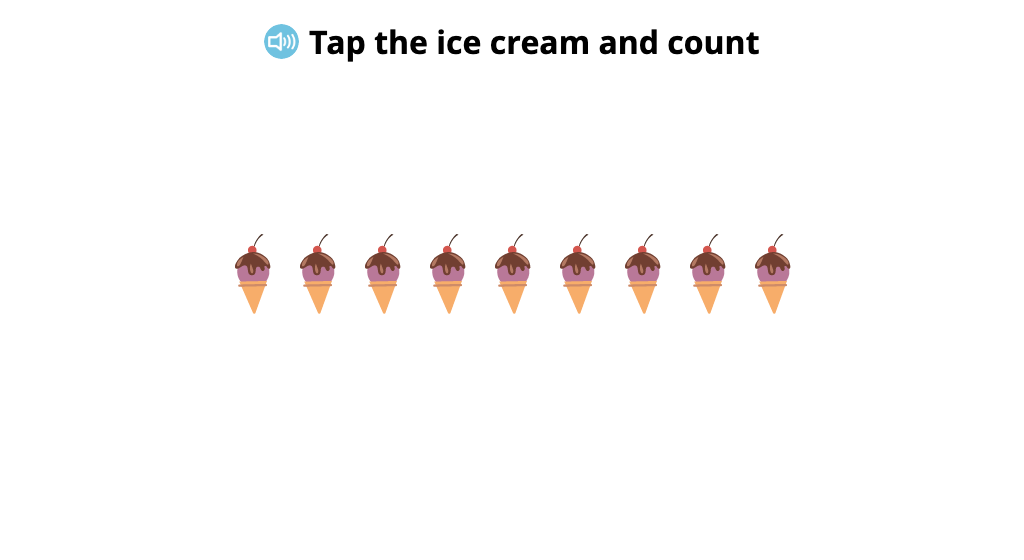#### Identify totals of up to 10 identical objects

Practice counting. Count up to 10 identical objects that are in a row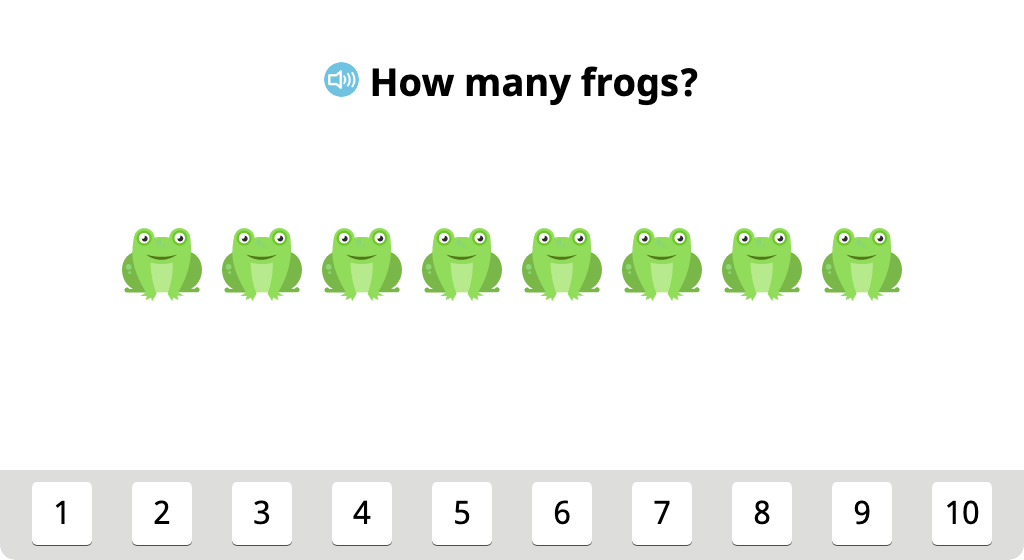#### Align groups of identical objects and identify the total

See that it is easier to count if you line up the objects in a row first. Align up to 10 objects in a box, then count them and click on the number that tells how many there are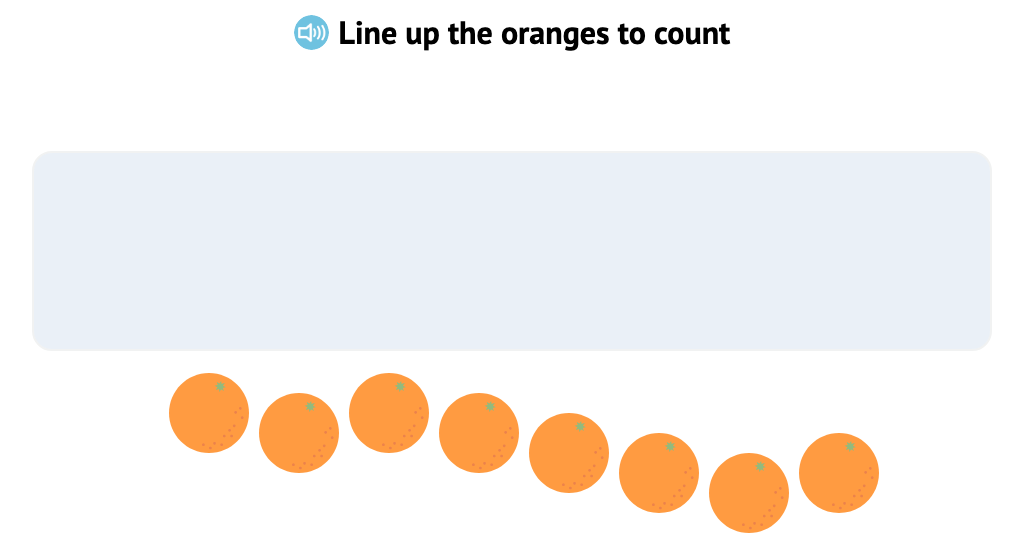#### Count identical objects in two rows from left to right, top to bottom

Practice counting up to 10 objects that are placed in two rows by counting left to right and top to bottom### Topic H: Matching One Numeral with up to 10 Objects

Students work with groups of identical objects to split totals in different ways (a precursor to learning fact families). They count objects in different alignments, including circular and two rows. In the final exercise, they see that when objects are rearranged, the total remains the same.

## MODULE 4. Comparison of Length, Weight, Capacity, and Numbers to 5

### Topic A: Comparison of Length

Students are introduced to the terms "taller," "higher," and "about the same" regarding height and length. They work with familiar objects to make determinations about height or weight, eventually manipulating objects to create the desired height or weight.

#### Determine which of two objects is taller

Practice with the words "taller" and "shorter." Place two objects next to each other, then click the taller object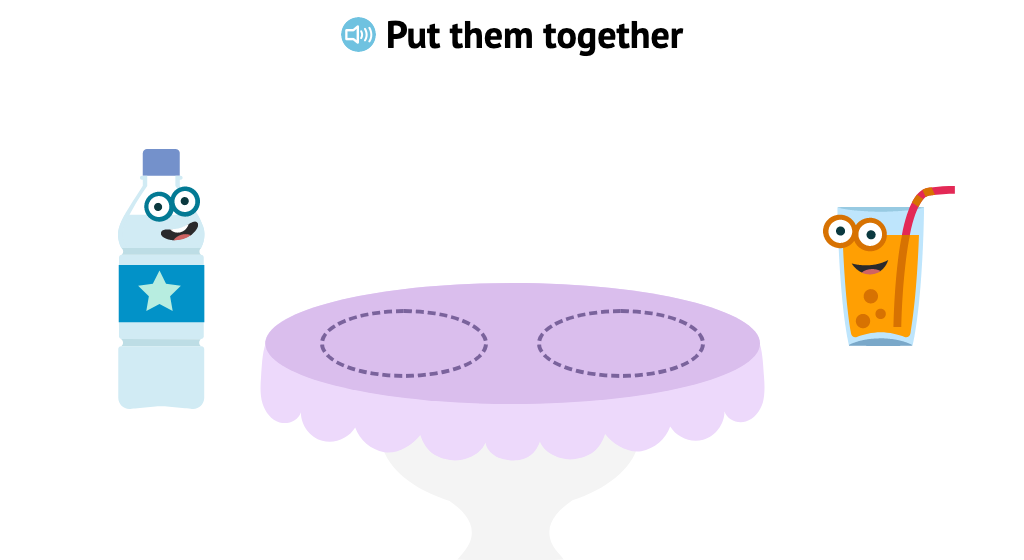#### Determine which of two objects is longer or shorter

Practice with the words "longer" and "shorter." Place two objects next to each other, then click the longer or shorter object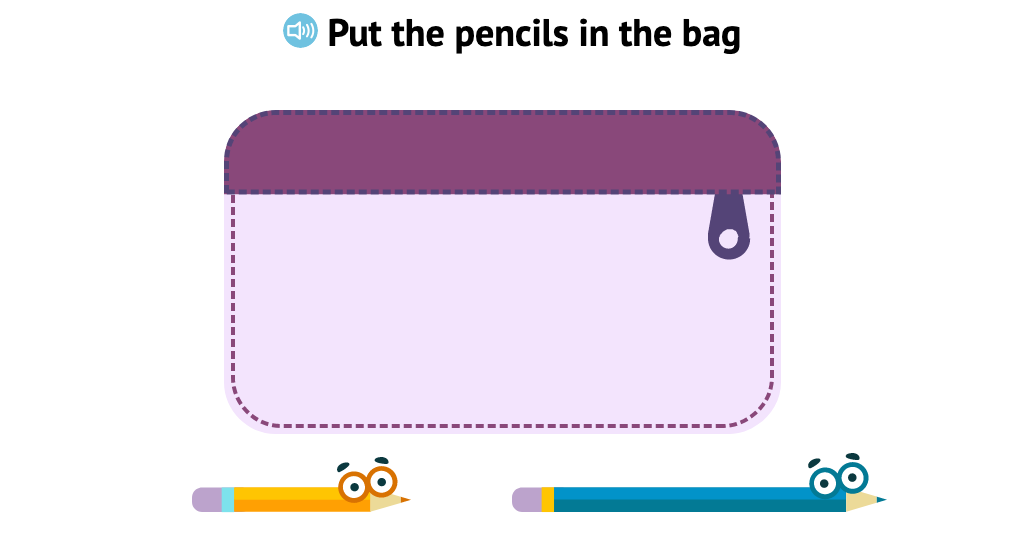#### Identify two objects that are about the same height

Practice identifying objects that are about the same height. Look at two pairs of objects. Choose the pair of objects that are about the same height. Then look at three objects and choose the two objects that are about the same height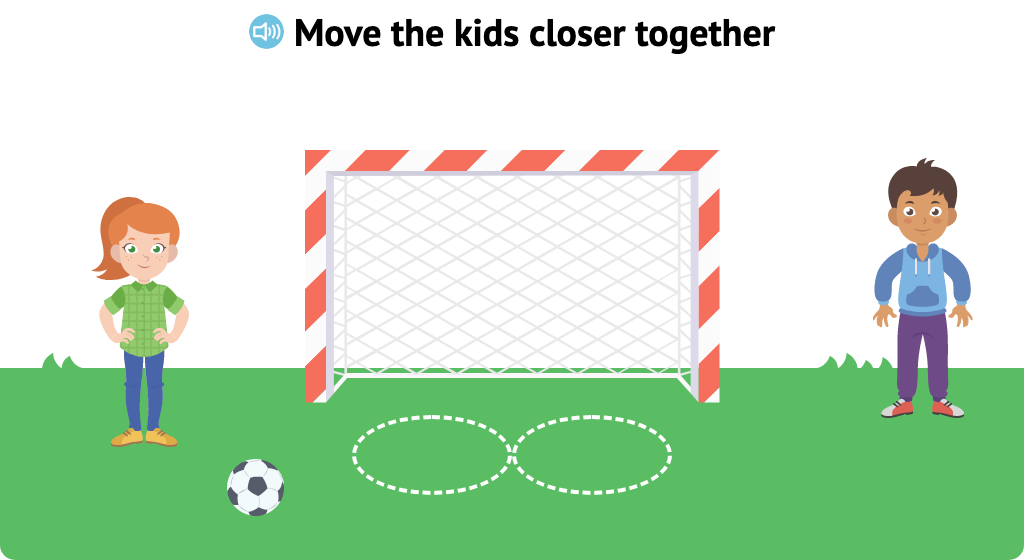#### Identify two objects that are about the same length

Look at three objects, and choose the two objects that are about the same length. Then show where to cut one ribbon to make it about the same length as another ribbon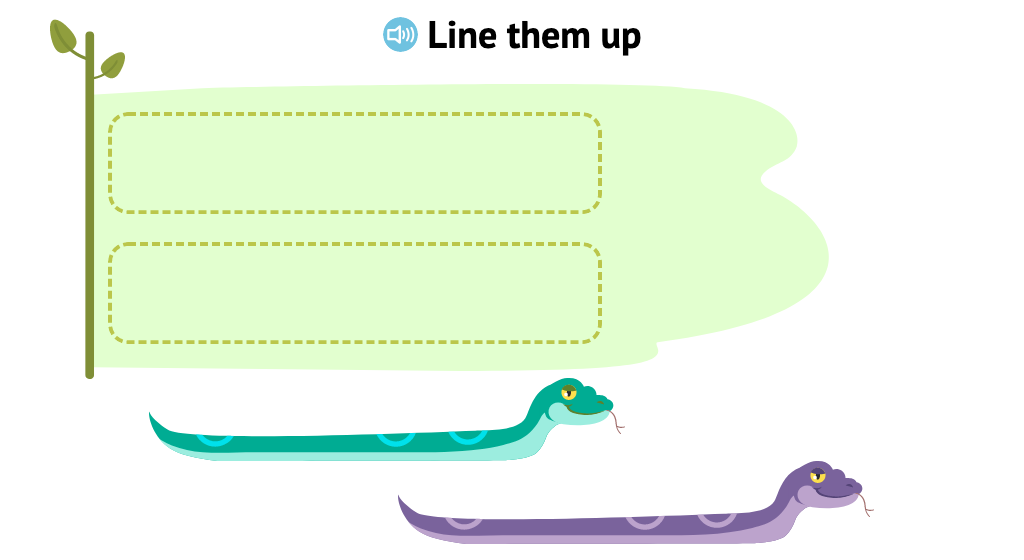#### Identify an object that is about the same length or height as a given object

Practice comparing length and height. Look at three objects and choose the one that is about the same length or height as a given object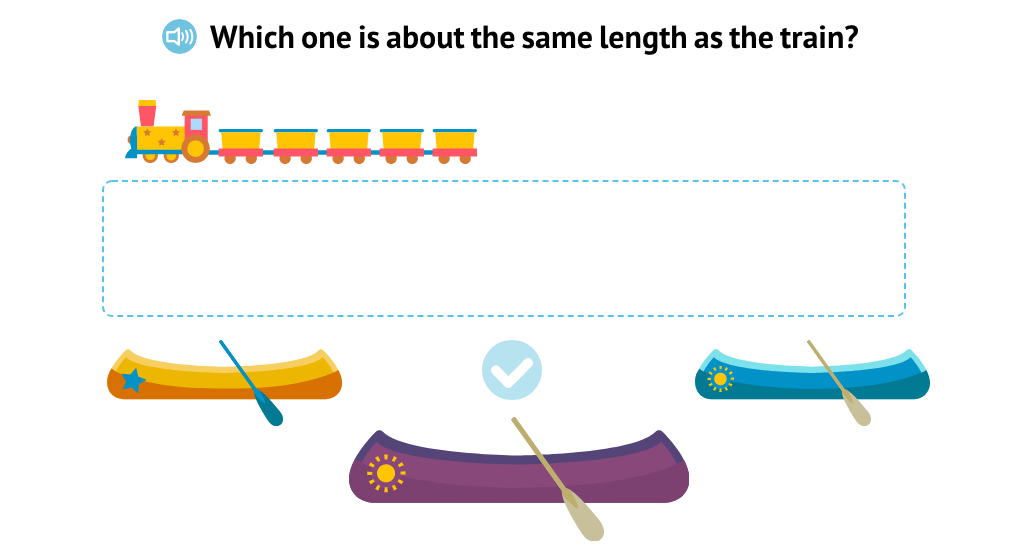#### Add cars or take them away to make a train the same length as a given train

Practice making trains of the same length. Add train cars or take train cars away to make a train the same length as a given train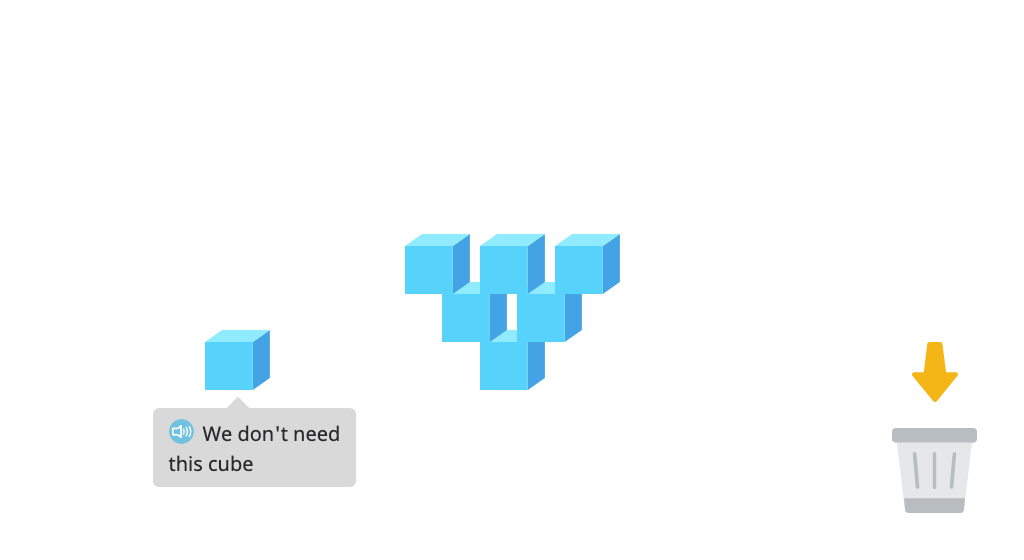#### Add cubes or take them away to make a tower the same height as a given tower

Build a tower with cubes to make a tower that is the same height as another tower. Then take cubes away from a tower until it is the same height as another tower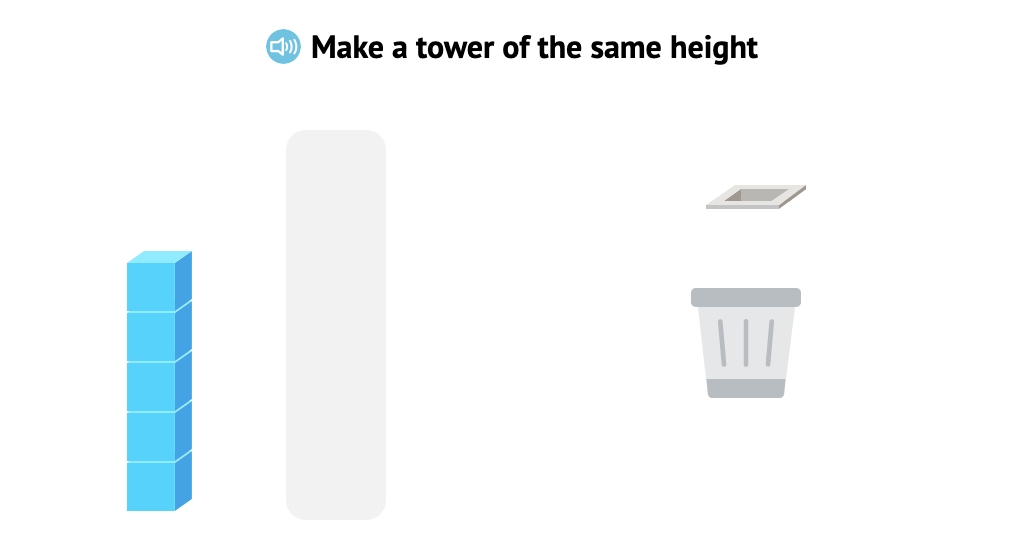### Topic B: Comparison of Weight

Students are introduced to the concept of weight, including the terms "lighter" and "heavier." Students will compare familiar objects to make determinations about weight.

### Topic C: Identifying Objects That Are First and Last

Students are introduced to the terms "first" and "last," then use this knowledge to identify which items are first and last in a series of 5 or less. Then, students are introduced to the concept of one-to-one correspondence.

### Topic D: Comparing Sets

Students are introduced to the concepts of "more," "less," "same," and "different." Given two sets of 5 or less objects, students will compare the number of items in each group and identify which shows more or less, or same or different amounts.

#### Determine which of two sets shows "more"

Students are introduced to the concept of "more," given two sets of objects. Students will identify which of two sets has more items. Sets contain 5 or less items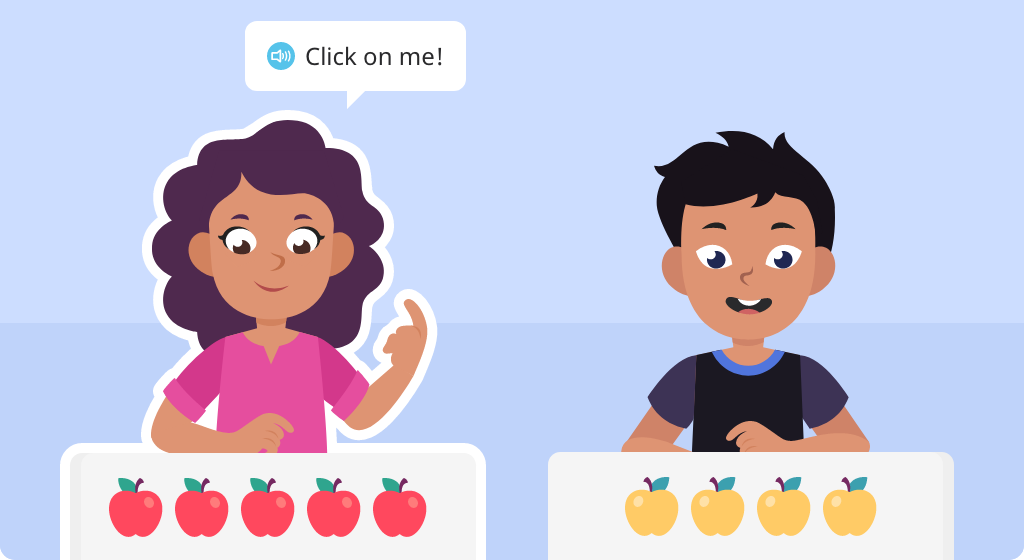#### Determine which of two sets shows "fewer"

Students are introduced to the concept of "fewer," given two sets of objects. Students will identify which of two sets has fewer items. Sets contain 5 or less items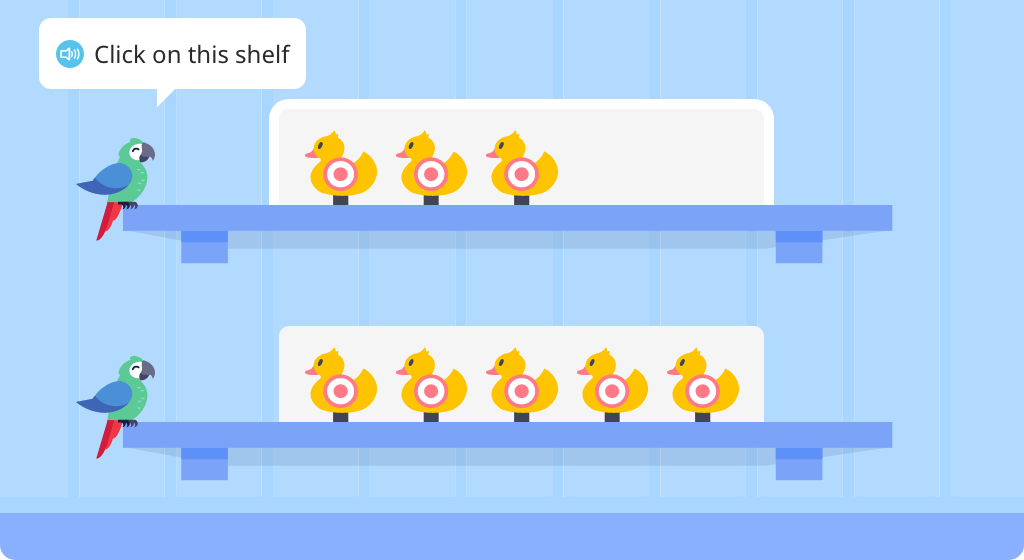#### Identify the number of cubes in two given stacks and compare them

Given two rods of 5 or less base-10 cubes to compare, students will determine which stack has more or less. Students will then count the number of cubes in each rod. Students are thereby introduced to the concept of comparing numbers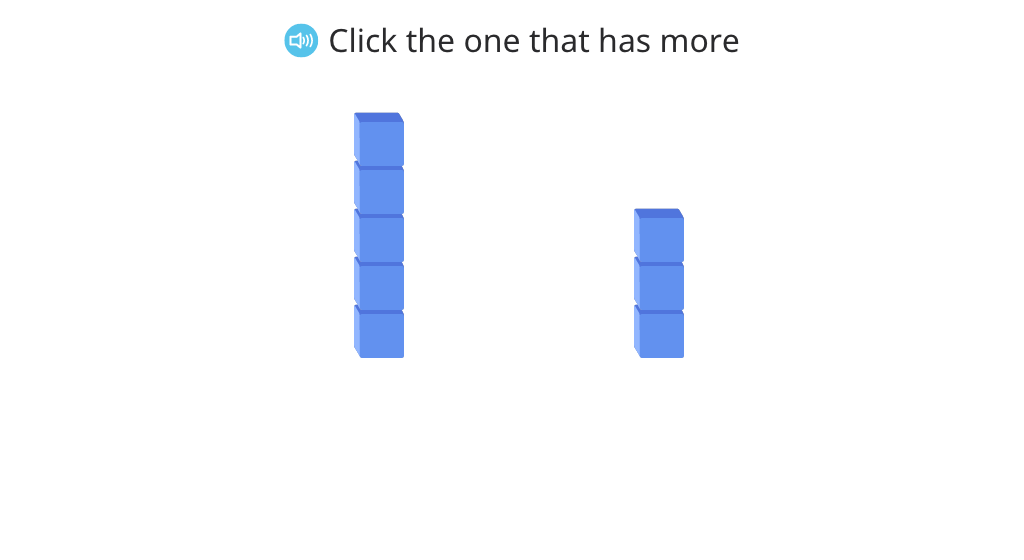#### Begin to understand the concepts of "same" and "different" amounts of objects

Students are introduced to the concept of "same," or equal, amounts of objects. Given two rods of 5 or less base-10 cubes to compare, students will determine if the two rods show same or different amounts of cubes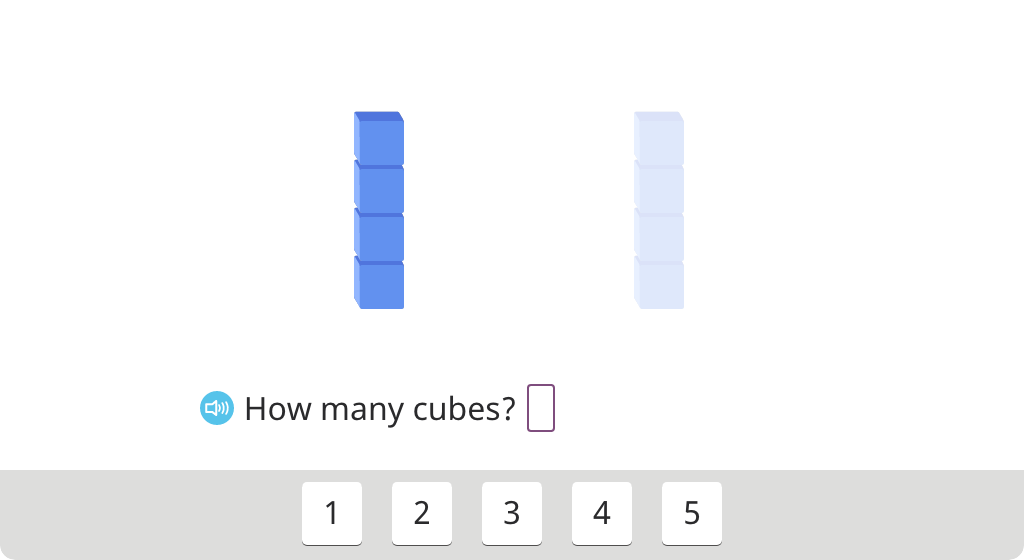#### Determine if two sets of base-10 cubes show the same amount

Given two rods of 5 or less base-10 cubes, students will compare the sets and determine if they do or do not show the same amount of cubes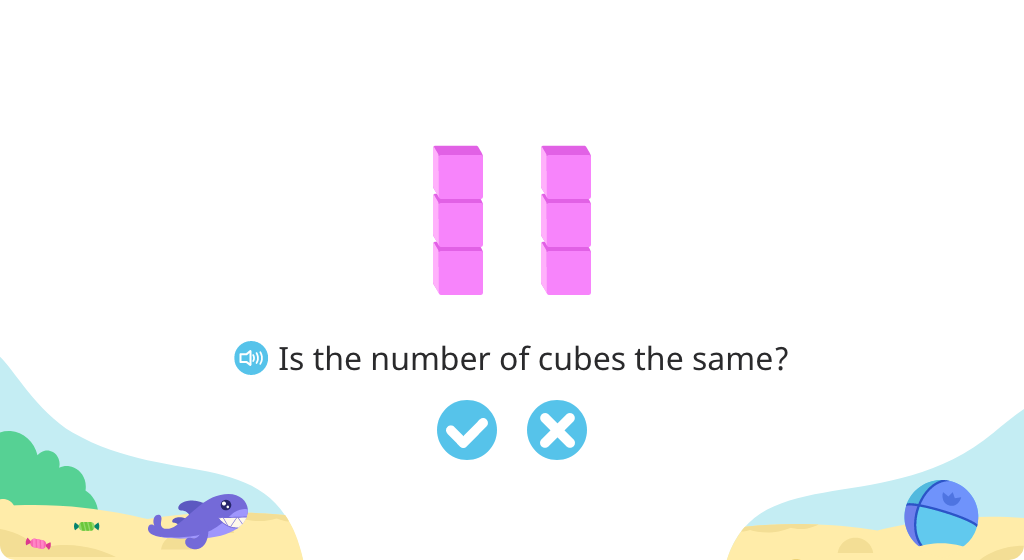## MODULE 5 Addition and Subtraction Stories

### Topic A: Contextualizing Addition Stories to Solve

Students count identical objects aligned in a row. Then additional objects, identical except for the color, are added to the row. Students count those objects and then determine the total.

### Topic B: Contextualizing Subtraction Stories to Solve

Students count identical objects aligned in a row. Then some of the objects are taken away (balloons pop, pears are eaten, etc.). Students count the remaining objects to determine the difference.

### Topic C: Decontextualizing Addition Stories to Solve Using Fingers, Objects, and Drawings

Students move toward more abstract thinking by using cubes to represent real objects (birds, balloons, apples, etc.). They build understanding that the number of cubes represents the objects with 1:1 matching and can be used in determining totals in simple addition stories.

#### Match cubes 1:1 with objects aligned in a row (Level 1)

Match cubes one-to-one with up to 4 objects. This will help students model story problems using cubes as a model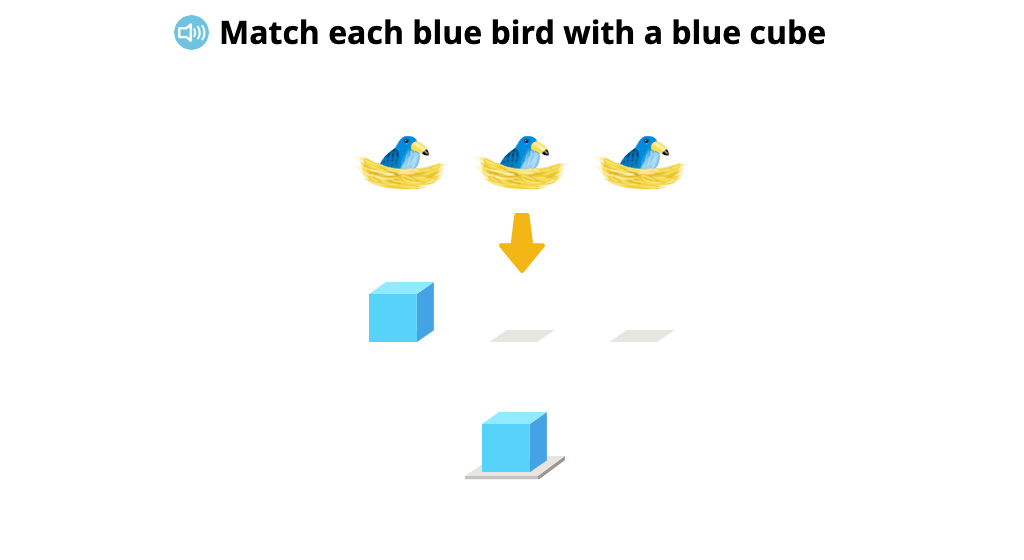#### Match cubes 1:1 with objects aligned in a row (Level 2)

Practice modeling a situation with cubes. Look at a picture of up to 4 objects. Place the correct number of cubes to model the number of objects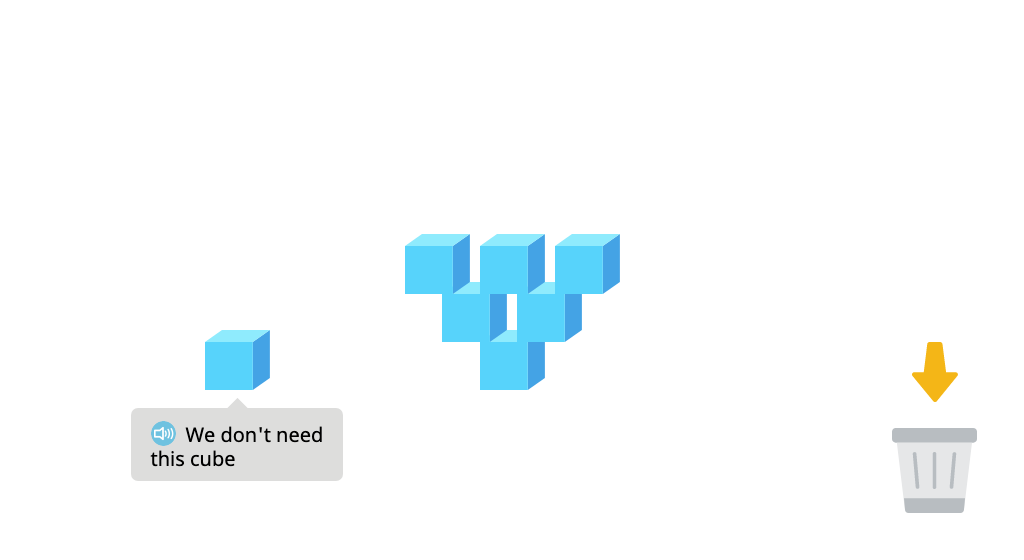#### Determine the number of objects represented by cubes

Practice modeling a situation with cubes. Look at a picture of up to 4 objects. Place the correct number of cubes to model the number of objects. Then, the objects will be hidden. Look at the number of cubes, and tell how many objects are hidden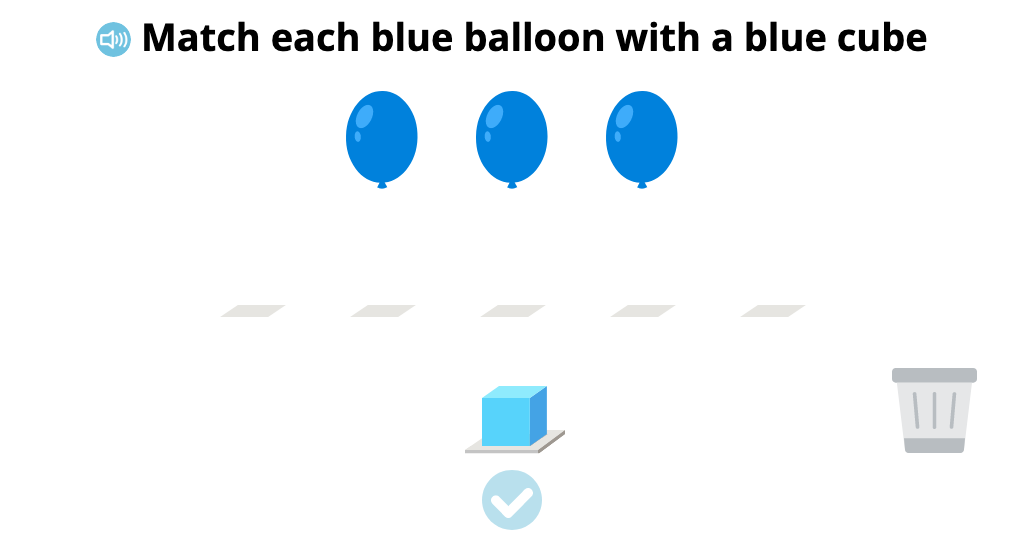#### Match cubes 1:1 with objects aligned in a row (Level 3)

Practice one-to-one correspondence and modeling. Model different colored objects with different colored cubes. Make sure the number of cubes matches the number of objects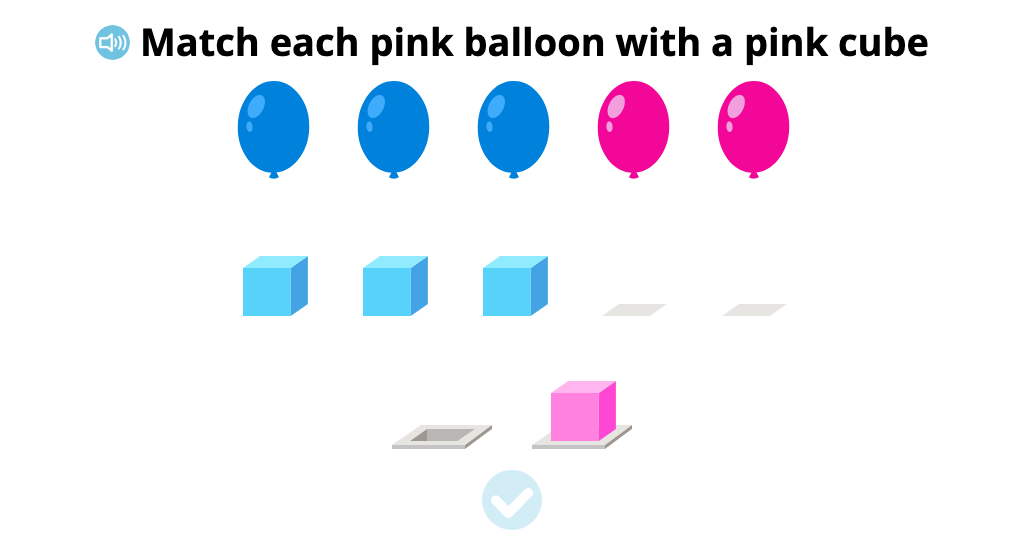#### Determine the number of objects represented by cubes in an addition scenario (Level 1)

Model addition situations with cubes. Use a different colored cubes for different colored objects. Then, the objects will be hidden. Find how many objects there are altogether by counting the cubes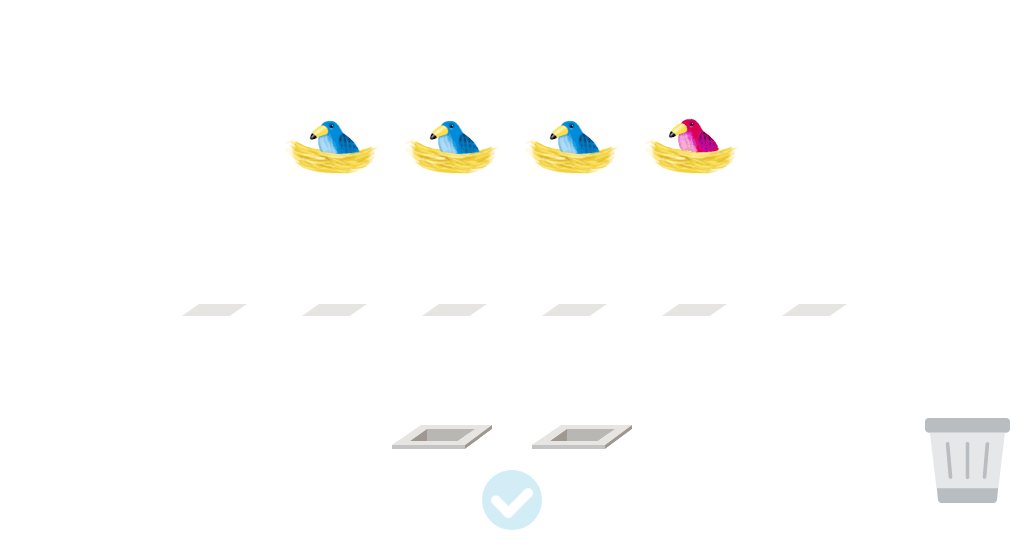#### Determine the number of objects represented by cubes in an addition scenario (Level 2)

Practice modeling with cubes. Listen to a description telling a number of objects and model the number of objects using cubes. Then, set up addition problems with sums to 5 by modeling the two addends with cubes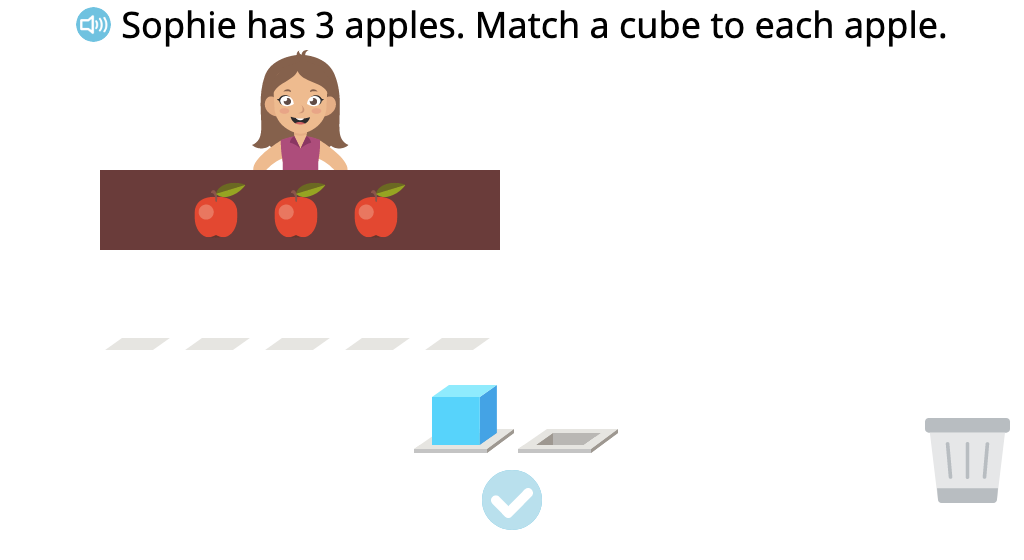#### Determine the total of two groups of objects based on their representation in cubes

Use cubes to model an addition story problem. Then solve the problem by finding the total number of cubes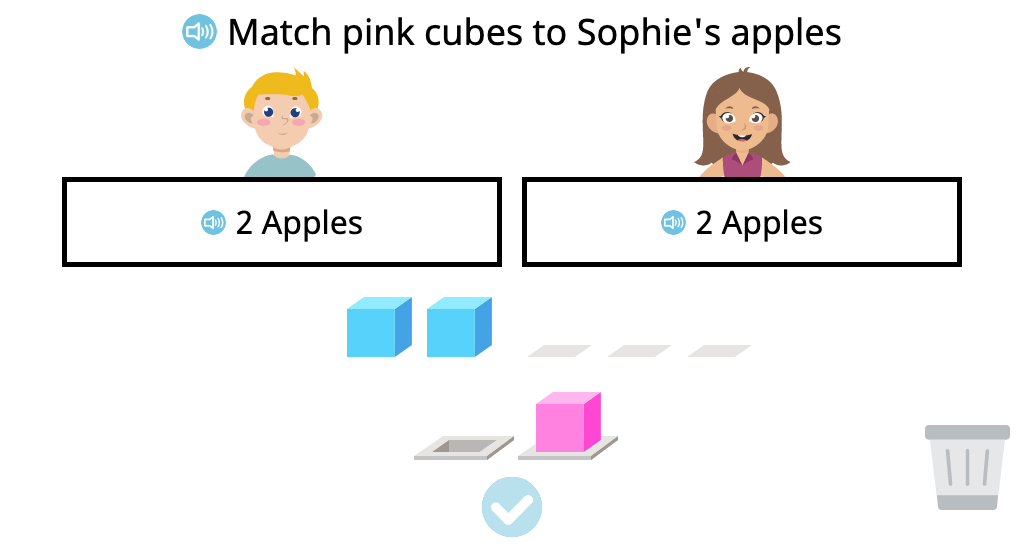### Topic D: Decontextualizing Subtraction Stories to Solve Using Fingers, Objects, and Drawings

Students move toward more abstract thinking by using cubes to represent real objects . They build understanding that the number of cubes represents the objects with 1:1 matching and can be used in determining totals in simple subtraction stories.